Journal DetailsFormat
Journal
First Published
01 Jan 2016
Publication timeframe
2 times per year
Languages
English
Copyright
© 2020 Sciendo

# Accuracy Problems of Numerical Calculation of Fractional Order Derivatives and Integrals Applying the Riemann-Liouville/Caputo Formulas

###### Accepted: 04 Nov 2015
Journal DetailsFormat
Journal
First Published
01 Jan 2016
Publication timeframe
2 times per year
Languages
English
Copyright
© 2020 Sciendo

In the paper there are presented and evaluated for effectiveness three methods of accuracy increase of fractional order derivatives and integrals computations for application with the Riemann-Liouville/Caputo formulas. They are based on the ideas of either transforming difficult integrand in the formulas to high-accuracy computations requirements of a applied method of numerical integration or adapting a numerical method of integration to handle with high-accuracy a difficult feature in the integrand. Additional accuracy gain is obtained by incorporating increased precision into computations. The actual accuracy improvement by applying presented methods is compared with the capabilities of wide range of available methods of integration.

#### MSC 2010

Introduction

Fractional calculus may be described as an extension of the concept of a derivative operator from integer order n to arbitrary order ν, where ν is a real or complex value or a complex valued function ν = ν(x,t) :

$d(n)dx(n)→d(ν)dx(ν).$$$\begin{array}{} \displaystyle \frac{{{d^{\left( n \right)}}}}{{d{x^{\left( n \right)}}}} \to \frac{{{d^{\left( \nu \right)}}}}{{d{x^{\left( \nu \right)}}}}. \end{array}$$

Beginnings of fractional calculus indeed date to the same time as classical calculus. However, the question of consequences of “taking square root of 1st derivative” arose in 1695  is not to be discussed for more than 100 years. Well known Riemann-Liouville definition of fractional derivative and integral was reported by Liouville in 1832 and by Riemann in 1876. Grünwald introduced the idea of fractional derivative as the limit of a sum in 1867 .

The most rapid interest grow of this new topic can be observed in the last 40 years. The first conference “International Conference of Fractional Calculus and Its Applications” entirely dedicated to it took place in 1974 at the University of New Heaven, Connecticut, USA . The first monograph dedicated to fractional calculus was published the same year .

From that time on, the topic won interest of scientists from various areas of mathematics, physics, chemistry, electrical engineering, economy, biological sciences. Initial works had a pure theoretical character. However, lately, can be noticed intensification of research on practical application of this tool in physics, technical, biological and economical sciences. In technical sciences, application of fractional calculus is mostly applied for areas of electrical engineering, electronics and control systems as well as image analysis and processing.

Fractional calculus requires extensive application of numerical methods, e.g. numerical integration. However, commonly applied numerical methods are not suitable for that purpose in their existing form.

Effectiveness of fractional derivatives and integrals practical applications can be increased by improving their computational accuracy. Thus, increasing computational accuracy of fractional order derivatives and integrals became a very important task to study for many computer scientists. Increasing it as high as possible and by using as little as possible hardware resources and time is a matter of aptly selected method, applied hardware, programming language and programming techniques in the process.

Growing popularity of computer as a tool for scientific research led to essential advancements of existing programming languages. C++ can be considered as best example in this context. C++ is a general purpose programming language, which enable hardware speed optimization. It is a flexible language with great versatility and it has huge function library which can be used for developing system software, e.g. operating systems, compilers, editors and data bases.

To be able to solve difficult numerical problem according to a set goal, a scientist has to make some crucial decisions regarding applied for that purpose hardware, programming tools and techniques. They include appropriate selection of computer programming languages because their abilities to process numerical data determine how accurate the solution will be.

The selection of uniform C++ equipped with standard mathematical library as a main programming tool is not enough nowadays to solve numerical problems with satisfactory accuracy.

Double precision arithmetic commonly applied in scientific numerical calculations has many flaws which influences negatively the accuracy of computations, e.g. limitations of number values which double precision variables can hold or no influence of programmer on mathematical operations rounding.

Arbitrary precision mathematical library GNU MPFR , built upon GNU GMP library  application enable any computation to be performed with any precision limited only by hardware, with either real or complex numbers. The library supports proper rounding of all mathematical operations as wel, which is crucial because most of inaccuracies, errors and limitations in scientific computations is caused by finite precision arithmetic and a lack of proper rounding of mathematical operations. The library has also at their disposal many ready to use functions, for example gamma function and reciprocal gamma function, which are extensively used for fractional order derivatives and integrals computations.

The Definitions of Fractional Order Derivatives and Integrals

English term the fractional calculus is misleading because it suggests that differentiation and integration orders may only assume non-integer values only. The more appropriate description of this branch of mathematics would be differentiation and integration of any order. In practice, both integration as well as differentiation order may assume irrational or rational, real or complex values , . Differentiation operator of non-integer order may be represented with a help of generalized operator $t0Dtν$$\begin{array}{} \displaystyle _{{t_0}}D_t^\nu \end{array}$ which is valid for operations of differentiation and integration. It is defined as follows

$$\begin{array}{} \displaystyle _{{t_0}}D_t^{\left( \nu \right)} = \left\{\begin{array}{*{20}{c}} {\frac{{{d^\nu }}}{{d{t^\nu }}}}&{{\rm{for }}\nu \gt 1}\\ 1&{{\rm{for }}\nu = 0}\\ {\int _{{t_0}}^t{{\left( {d\tau } \right)}^{ - \nu }}}&{{\rm{for }}\nu < 0,} \end{array}\right. \end{array}$$

in which:

ν is an order of differentiation and integration (it may assume as well real as complex values), t0, t are the interval limit of differentiation or integration.

Fractional derivative or integral is determined in [t0, t] interval, which narrows to a point in case of integer order. To differentiate between non-integer and integer orders, non-integer orders are commonly denoted by the Greek letters ν and μ and integer orders by the letters m and n.

The following definitions of fractional order differentiation and integration are the scope of the following paper:

Riemann-Liouville (RL) fractional derivative , 

$t0RLDt(ν)f(t)=(ddt)n1Γ(n−ν)∫t0tf(τ)(t−τ)ν−n+1dτ, for(n−1<ν$$\begin{array}{} \displaystyle _{{t_0}}^{RL}{\rm{D}}_t^{\left( \nu \right)}f\left( t \right) = {\left( {\frac{d}{{dt}}} \right)^n}\frac{1}{{\Gamma \left( {n - \nu } \right)}}\int_{{t_0}}^t {\frac{{f\left( \tau \right)}}{{{{\left( {t - \tau } \right)}^{\nu - n + 1}}}}} d\tau ,\;\;{\rm{for}}\left( {n - 1 < \nu < n} \right), \end{array}$$

in which n is an integer number n = ⌈ν⌉ and Γ(x) denotes Euler’s Gamma function.

Caputo (C) fractional derivative 

$0CDt(ν)f(t)=1Γ(n−ν)∫0tf(n)(τ)(t−τ)ν−n+1dτ, for(n−1<ν$$\begin{array}{} \displaystyle _0^C{\rm{D}}_t^{\left( \nu \right)}f\left( t \right) = \frac{1}{{\Gamma \left( {n - \nu } \right)}}\int_0^t {\frac{{{f^{\left( n \right)}}\left( \tau \right)}}{{{{\left( {t - \tau } \right)}^{\nu - n + 1}}}}} d\tau ,\;\;{\rm{for}}\left( {n - 1 < \nu < n} \right). \end{array}$$

Formulas (2) and (3) are related by

$t0RLDt(ν)f(t)=0CDt(ν)f(t)+∑k=0n−1tk−νΓ(k−ν+1)fk(0).$$$\begin{array}{} \displaystyle _{{t_0}}^{RL}D_t^{\left( \nu \right)}f\left( t \right) = _0^CD_t^{\left( \nu \right)}f\left( t \right) + \sum\limits_{k = 0}^{n - 1} {\frac{{{t^{k - \nu }}}}{{\Gamma \left( {k - \nu + 1} \right)}}} {f^k}\left( 0 \right). \end{array}$$

Riemann-Liouville fractional derivative equivalent to (7), , 

$t0RLDt(ν)f(t)=∑k=0n−1(t−t0)k−νf(k)(t0)Γ(k−ν+1)+1Γ(n−ν)∫t0tf(n)(τ)(t−τ)ν−n+1dτ, for(n−1<ν$$\begin{array}{} \displaystyle _{{t_0}}^{RL}{\rm{D}}_t^{\left( \nu \right)}f\left( t \right) = \sum\limits_{k = 0}^{n - 1} {\frac{{{{\left( {t - {t_0}} \right)}^{k - \nu }}{f^{\left( k \right)}}\left( {{t_0}} \right)}}{{\Gamma \left( {k - \nu + 1} \right)}}} + \frac{1}{{\Gamma \left( {n - \nu } \right)}}\int_{{t_0}}^t {\frac{{{f^{\left( n \right)}}\left( \tau \right)}}{{{{\left( {t - \tau } \right)}^{\nu - n + 1}}}}} d\tau ,\;{\rm{for}}\left( {n - 1 < \nu < n} \right). \end{array}$$

Riemann-Liouville fractional integral (RL) , 

$t0RLDt(−ν)f(t)=1Γ(ν)∫t0tf(τ)(t−τ)n−νdτ, for(n−1<ν$$\begin{array}{} \displaystyle _{{t_0}}^{RL}{\rm{D}}_t^{\left( { - \nu } \right)}f\left( t \right) = \frac{1}{{\Gamma \left( \nu \right)}}\int_{{t_0}}^t {\frac{{f\left( \tau \right)}}{{{{\left( {t - \tau } \right)}^{n - \nu }}}}} d\tau ,\;\;{\rm{for}}\left( {n - 1 < \nu < n} \right). \end{array}$$

Additionally, for comparison purposes there is also applied the Grünwald-Letnikov (GL) formula of fractional order backward difference/sum , , 

$t0GLDt(ν)f(t)=limh→01hν∑j=0⌊(t−t0)/h⌋(−1)j(νj)f(t−jh).$$$\begin{array}{} \displaystyle _{{t_0}}^{GL}D_t^{\left( \nu \right)}f\left( t \right) = \mathop {\lim }\limits_{h \to 0} \frac{1}{{{h^\nu }}}\sum\limits_{j = 0}^{\left\lfloor {\left( {t - {t_0}} \right)/h} \right\rfloor } {{{\left( { - 1} \right)}^j}} \left( \begin{array}{c} \nu \\ j \end{array} \right)f\left( {t - jh} \right). \end{array}$$

where t0,t is the integration interval.

Problems of Fractional Order Derivatives and Integrals Numerical Calculations

Fractional order derivatives and integrals computational formulas (3), (5) and (6) consist of ordinary integrals. However, the integrals are difficult to compute due to presence of singularity at the end of integration interval preceded by rapid values increase (stiffness).

Each integrand included in the formulas can be divided into a kernel and a function, i.e.

$t0RLDt(−ν)f(t)=1Γ(ν)∫t0t(t−τ)ν−n︸kernel f(τ)dτ, for(n−1<ν$$\begin{array}{} \displaystyle _{{t_0}}^{RL}{\rm{D}}_t^{\left( { - \nu } \right)}f\left( t \right) = \frac{1}{{\Gamma \left( \nu \right)}}\int_{{t_0}}^t {\underbrace {{{\left( {t - \tau } \right)}^{\nu - n}}}_{{\rm{kernel}}}} \;f\left( \tau \right)d\tau ,\;\;{\rm{for}}\left( {n - 1 < \nu < n} \right). \end{array}$$

Plot of the kernel of the integrand is presented in Fig. 1. Gray area denotes integral to compute.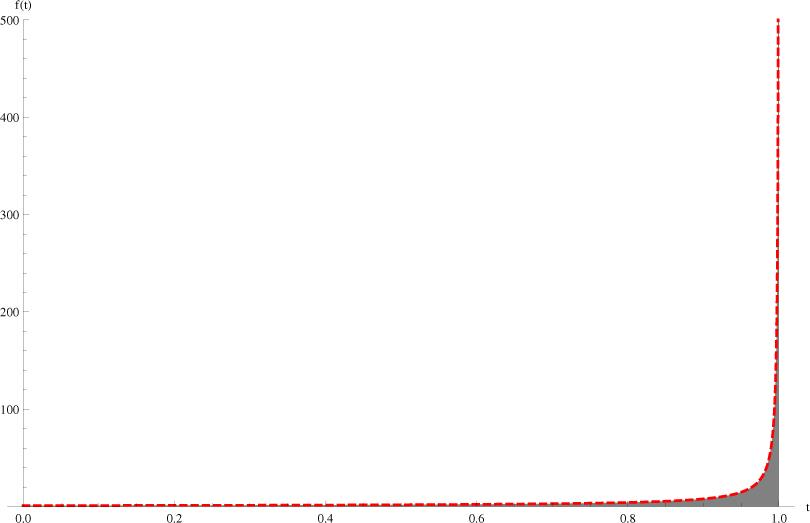Plot of the kernel of the integrand in the formulas (3), (5) and (6).

Stiffness of the integrand can increase or decrease according to a value of power and a character of a function.

This characteristics of the kernel determines accuracy of computations.

To compute fractional order derivatives and integrals with help of formulas (3), (5) and (6) there can be applied commonly used methods of numerical integration, e.g. Midpoint Rule, Trapezoidal Rule as well as Gauss-Legendre and Gauss-Kronrod Quadratures (more informations on the methods can be found in  and ).

Due to the singularity problem in the integrand there can be applied some techniques advised in the literature , , , : (i) Increasing of subintervals amount, (ii) Exclusion of singularity from integration, (iii) Stretching difficult section and application of adaptive algorithm to it (an amount of subintervals adjusted to variability of an integrand) and (iv) Integrating of reciprocal function.

Unfortunately excluding the singularity from the integration decreases in this case accuracy even more because area which contributes to integral value at most proceeds it directly.

Stretching the difficult section even many times and using an appropriately increased amount of subintervals does not effect accuracy satisfyingly. The end section of the integrand is too stiff for the listed methods of numerical integration.

Reciprocal function integration can be applied to simple, one-valued functions only. Therefore, it may not be applied as a general solution of the problem.

Another solution can be an application of numerical methods which have been developed or modified particularly for application with (3), (5) and (6). There exist only a few such methods. They are based on: (i) Extrapolation , (ii) Trapezoidal Rule , (iii) Modified Trapezoidal Rule for Caputo derivatives only , (iii) Mollification techniques , (iv) Chebyshev polynomials (for fractional integrals only) .

However, the only one algorithm which can be considered as significant is the one based on the Trapezoidal Rule.

Diethelm removed analytically the singularity from the integrand by applying integrating by parts and calculating some quadrature coefficients. Resulting accuracy of fractional order derivatives and integrals computations can be additionally improved by application of the Richardson Extrapolation.

Diethelm’s method has been redesigned by Odibat for application for Caputo fractional derivatives only . His modifications are of minor importance and did not increased overall accuracy capabilities of the method.

Maximum of available computational accuracy of fractional order derivatives and integrals calculations applying formulas (5) and (6) and presently available methods of numerical integration is presented in the tables 1-3.

D(1/2)f(t), f(t) = t, t ∈ (0,1), relative error in %

NGLNCmDietOdiba
88.1110.680.00040.0024
154.257.810.00040.0023
213.026.60.00040.0023
611.033.880.00040.0022
3000.211.750.00020.0021
6000.111.240.00020.0021
10000.060.960.00020.0021

D(1/2)f(t), f(t) = e−t, t ∈ (0,5), relative error in %

NGLNCm
861.8713.52
1529.325.88
2120.174.12
616.541.81
3001.30.76
6000.650.53
10000.390.41

D(1/2)f(t), f(t) = sin(t), t ∈ (0,2π), relative error in %

NGLNCmDietOdiba
8130.0345.4217.675.78
1566.1433.647.953.46
2146.0928.775.182.94
6116.117.841.532.94
3005.029.350.622.43
6003.677.290.562.35
10003.146.180.552.35

Applied methods include the Grünwald-Letnikov method (GL) (7), Newton-Cotes Midpoint Rule (NCm) and modified Trapezoidal Rule by Diethelm (Diet) and Odibat (Odiba). There are not included any results for Gauss Quadratures because they are not suitable for this kind of calculations (relative error exceeding 90%). The same applies to Diethelm’s and Odibat’s method applied for fractional derivative of the exponential function.

The programs for this part has been created by the author using algorithms described in the freely available papers and literature of the subject.

Computational accuracy measure assumed in the following section is percentage relative error. Computer programs are written using C++ with double precision and complied applying GNU GCC compiler.

Computer configuration include Intel i7 processor, 8GB of RAM and Linux Ubuntu 14.04 LTS 64-bit operating system.

The results presented in the Tables 1-3 suggest that increasing an amount of subintervals N does not improve computational accuracy satisfyingly.

The method by Diethelm and its modification by Odibat enable to calculate fractional derivatives and integrals of some orders and of some functions with accuracy limited at best to 3-4 decimal places.

Therefore, the conclusion is that none of the presently available methods can be considered as a method of general use which assures computations of fractional derivatives and integrals with high accuracy independently from order, interval and type of the function.

Three Approaches to the Accuracy Increase for Riemann-Liouville and Caputo Formulas

Conducted survey suggests that to calculate fractional order derivatives and integrals with higher accuracy than presently is possible either an applied method of numerical integration must be adapted to handle with high-accuracy an integrand with the difficult feature or an integrand must be transformed to fit into a numerical method’s of integration requirements for high accuracy of computations.

In the next three subsections there are presented various approaches to the subject of accuracy increase of fractional order derivatives and integrals computations for application with the Riemann-Liouville and Caputo formulas.

Analytical Integrand Transformation Prior Numerical Integration

A method of transforming an integrand is analytical integrand transformation via independent variable substitution. It has to be conducted prior numerical integration.

Its rules are well known from analytical integration. However, this time instead of transforming an integrand into one which is analytically easier integrable, the goal is to obtain an integrand with a shape which fits into requirements of a selected method of numerical integration for high accuracy of computations.

Application of variable change

$1−τ→e1−1/u$$$\begin{array}{} \displaystyle 1 - \tau \to {e^{1 - 1/u}} \end{array}$$

in kernel of formula (5) and (6) removes singularity from the integrand and it makes it smoother.

For an example function f(t) = 1(t), t0 = 0,t = 1 and fractional order of integral ν = 0.5 we calculate

$∫01(1−τ)−0.5︸g(τ)dx=|1−τ=e1−1/u−dτ=e1−1/u⋅1u2dτ=(e1−1/u)⋅1u2|=…=…∫01(e1−1/u)0.5⋅1u2︸G(u)du.$$$\begin{array}{} \displaystyle \int_{0}^{1} \underbrace{\left ( 1-\tau \right )^{-0.5}}_\text{g\left(\tau\right)}dx & = \begin{vmatrix} 1-\tau = e^{1-1/u} \\ -d\tau = e^{1-1/u}\cdot\frac{1}{u^{2}} \\ d\tau = \left ( e^{1-1/u} \right ) \cdot\frac{1}{u^{2}} \end{vmatrix} = \dots \nonumber \\ & = \dots \int_{0}^{1} \underbrace{\left ( e^{1-1/u} \right )^{0.5}\cdot \frac{1}{u^{2}}}_\text{G\left(u\right)} du. \end{array}$$

$τ=1−e1−1/u,u=11−ln(1−τ).$$$\begin{array}{} \displaystyle \begin{array}{*{20}{l}} {\tau = 1 - {e^{1 - 1/u}},}\\ {u = \frac{1}{{1 - ln\left( {1 - \tau } \right)}}.} \end{array} \end{array}$$

Transformation (9) and integration interval change (10) is presented in Fig. 2.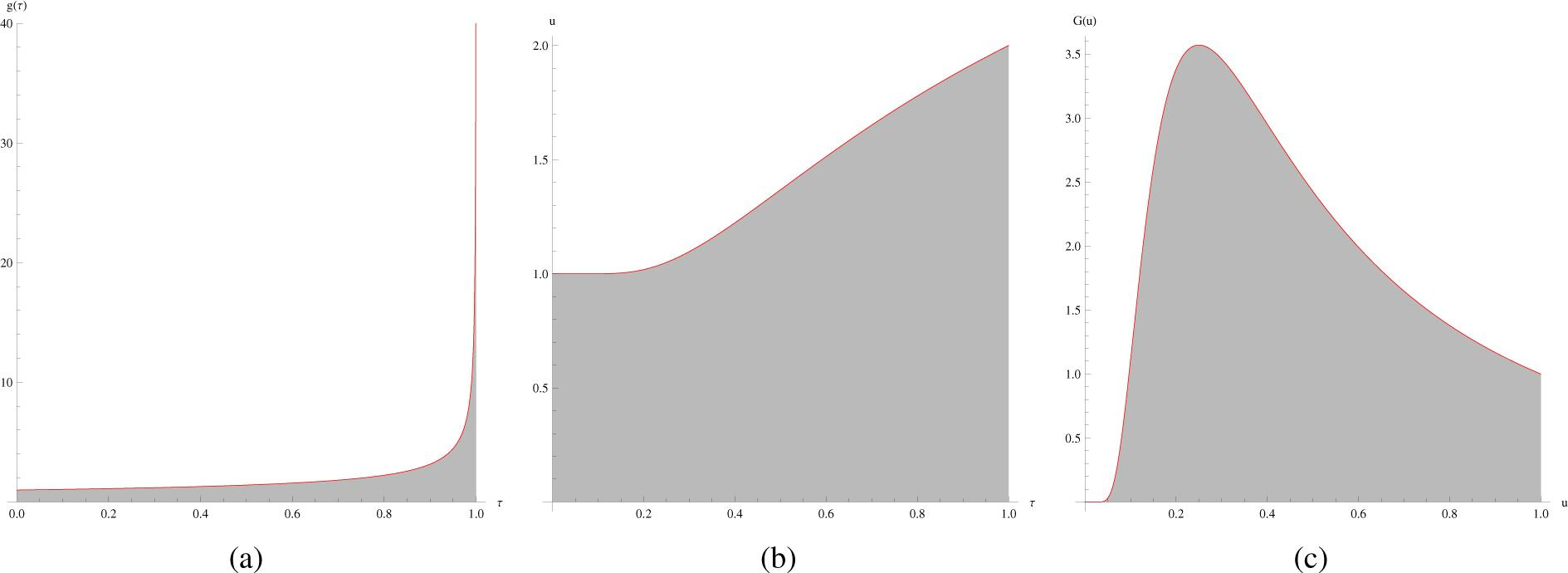(a) Graph of the original integrand g(τ), (b) graph of transformed variable τ into u and (c) transformed integrand g(τ) into G(u).

Transformation (9) is now applied for practical accuracy increase using two periodic functions:

$f(t)=sin(t), t∈(0,2π),$$$\begin{array}{} \displaystyle f\left( t \right) = \sin \left( t \right),\;t \in \left( {0,2\pi } \right), \end{array}$$

$f(t)=1.5cos(2t)+2.2cos(4t), t∈(0,2π).$$$\begin{array}{} \displaystyle f\left( t \right) = 1.5\cos \left( {2t} \right) + 2.2\cos \left( {4t} \right),\;t \in \left( {0,2\pi } \right). \end{array}$$

Fractional order integration formula (6) for the function (11) and order (−ν) assumes the following form

$0RLD2π(−ν)sin(t)=1Γ(ν)∫02π(e2π−2π/u)ν2πu2sin(2π−e2π−2π/u)du$$$\begin{array}{} \displaystyle _0^{RL}{\rm{D}}_{2\pi }^{\left( { - \nu } \right)}\sin \left( t \right) = \frac{1}{{\Gamma \left( \nu \right)}}\int_0^{2\pi } {{{\left( {{e^{2\pi - 2\pi /u}}} \right)}^\nu }} \frac{{2\pi }}{{{u^2}}}\sin \left( {2\pi - {e^{2\pi - 2\pi /u}}} \right)du \end{array}$$

and for the function (12) assumes the form

$0RLD2π(−ν)1.5cos(2t)+2.2cos(4t)=1Γ(ν)∫02π(e2π−2π/u)ν2πu2 +1.5cos(2(2π−e2π−2π/u)) +2.2cos(4(2π−e2π−2π/u))du.$$$\begin{array}{} \displaystyle _0^{RL}{\rm{D}}_{2\pi }^{\left( { - \nu } \right)}1.5\cos \left( {2t} \right) + 2.2\cos \left( {4t} \right) = \frac{1}{{\Gamma \left( \nu \right)}}\int _0^{2\pi }{\left( {{e^{2\pi - 2\pi /u}}} \right)^\nu }\frac{{2\pi }}{{{u^2}}}\, + 1.5\cos \left( {2\left( {2\pi - {e^{2\pi - 2\pi /u}}} \right)} \right)\, + 2.2\cos \left( {4\left( {2\pi - {e^{2\pi - 2\pi /u}}} \right)} \right)du. \end{array}$$

The accuracy of computations are presented in Figs 3(a) and 3(b). Due to similar performance of the methods for all three evaluated orders ν = 0.2,0.5,0.8, there are presented only plots for fractional order integrals of order ν = −0.5 and derivatives of order ν = 0.5.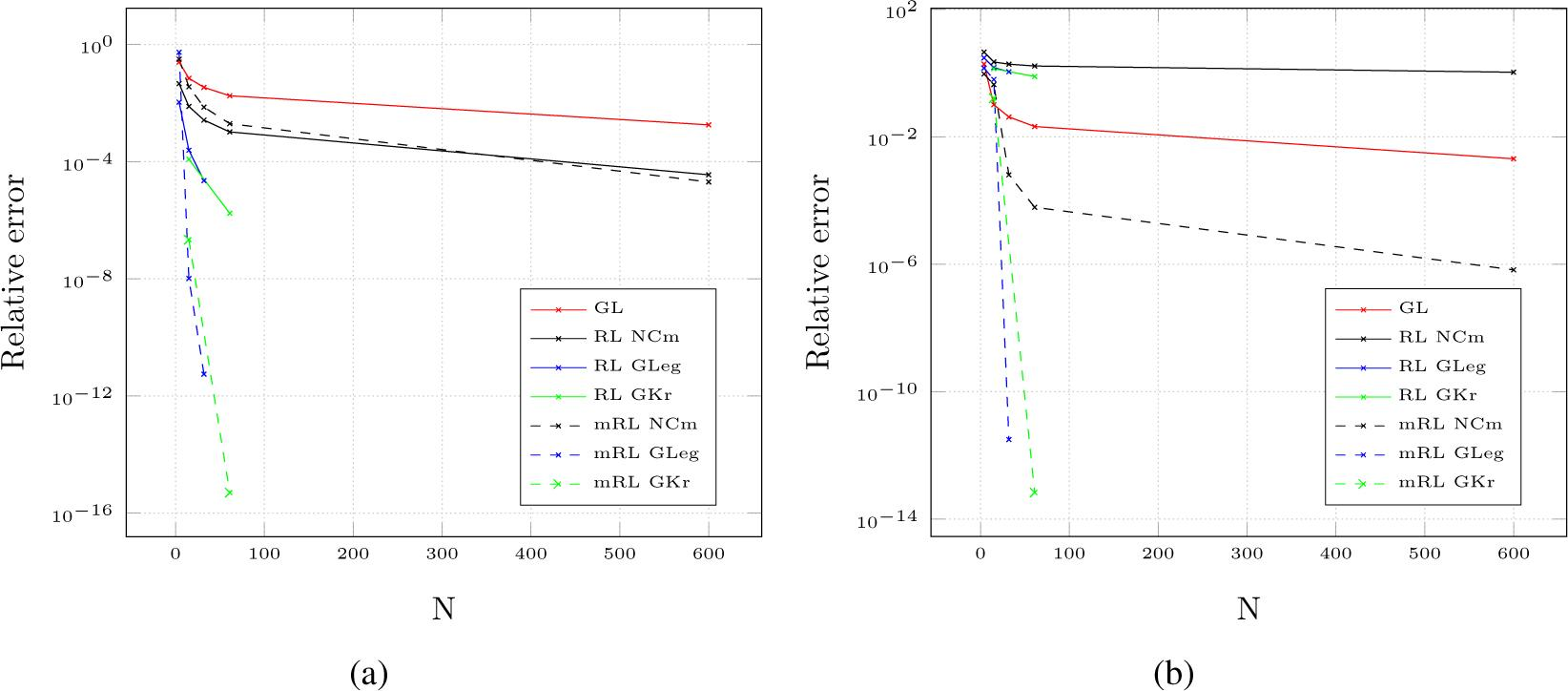Computational accuracy of fractional integral of order ν = −0.5 for the function (11)(a) and (12)(b) applying unmodified(RL ...) and modified(mRL ...) formula (6).

For accuracy increase evaluation there are applied well known quadratures. They are considered as highly accurate and efficient if an integrand fulfills their requirements. The methods include: Newton-Cotes Midpoint Rule (NCm), Gauss-Legendre Quadrature (GLeg), Gauss-Kronrod Quadrature (GKr), Gauss-Laguerre Quadrature (GLag), Gauss-Jacobi Quadrature (GJ).

The Grünwald-Letnikov formula (GL) (7) is applied for comparison purposes. Although it operates strictly on a integrated function’s values and can not be tested on transformed integrand, its accuracy can be considered as a point of reference.

Exact values required for error computation are calculated using analytical formulas to be found in ,  and . If a formula is not to be found, there is applied a novel approach to the accuracy assessment of fractional order derivatives and integrals using generalized fractional operators concatenation rules .

In the plots with results, accuracy of integration applying unchanged integrands are denoted with prefix RL, e.g. RL GKr and with modifications applied to the integrand - with prefix mRL, e.g. mRL GKr.

For fractional derivatives of order (ν), differentiation formula (5) for the function (11) assumes the following form

$0RLD2π(ν)sin(t)=(2π−ν)sin(0)Γ(1−ν)+1Γ(1−ν)∫02π(e2π−2π/u)ν2πu2cos(2π−e2π−2π/u)du$$$\begin{array}{} \displaystyle _0^{RL}{\rm{D}}_{2\pi }^{\left( \nu \right)}\sin \left( t \right) = \frac{{\left( {2{\pi ^{ - \nu }}} \right)\sin \left( 0 \right)}}{{\Gamma \left( {1 - \nu } \right)}} + \frac{1}{{\Gamma \left( {1 - \nu } \right)}}\int_0^{2\pi } {{{\left( {{e^{2\pi - 2\pi /u}}} \right)}^\nu }} \frac{{2\pi }}{{{u^2}}}\cos \left( {2\pi - {e^{2\pi - 2\pi /u}}} \right)du \end{array}$$

and for the function (12) assumes the form

$0RLD2π(ν)1.5cos(2t)+2.2cos(4t)=(2π−ν)1.5cos(0)+2.2cos(0)Γ(1−ν)+1Γ(1−ν)∫02π(e2π−2π/u)ν2πu2(−3sin(2(2π−e2π−2π/u)))−8.8sin(4(2π−e2π−2π/u))du.$$$\begin{array}{} \displaystyle _0^{RL}{\rm{D}}_{2\pi }^{\left( \nu \right)}1.5\cos \left( {2t} \right) + 2.2\cos \left( {4t} \right) = \frac{{\left( {2{\pi ^{ - \nu }}} \right)1.5\cos \left( 0 \right) + 2.2\cos \left( 0 \right)}}{{\Gamma \left( {1 - \nu } \right)}} + \frac{1}{{\Gamma \left( {1 - \nu } \right)}}\int _0^{2\pi }{\left( {{e^{2\pi - 2\pi /u}}} \right)^\nu }\frac{{2\pi }}{{{u^2}}}\left( { - 3\sin \left( {2\left( {2\pi - {e^{2\pi - 2\pi /u}}} \right)} \right)} \right) - 8.8\sin \left( {4\left( {2\pi - {e^{2\pi - 2\pi /u}}} \right)} \right)du. \end{array}$$

The accuracy of computations are presented in Figs 4(a) and 4(b).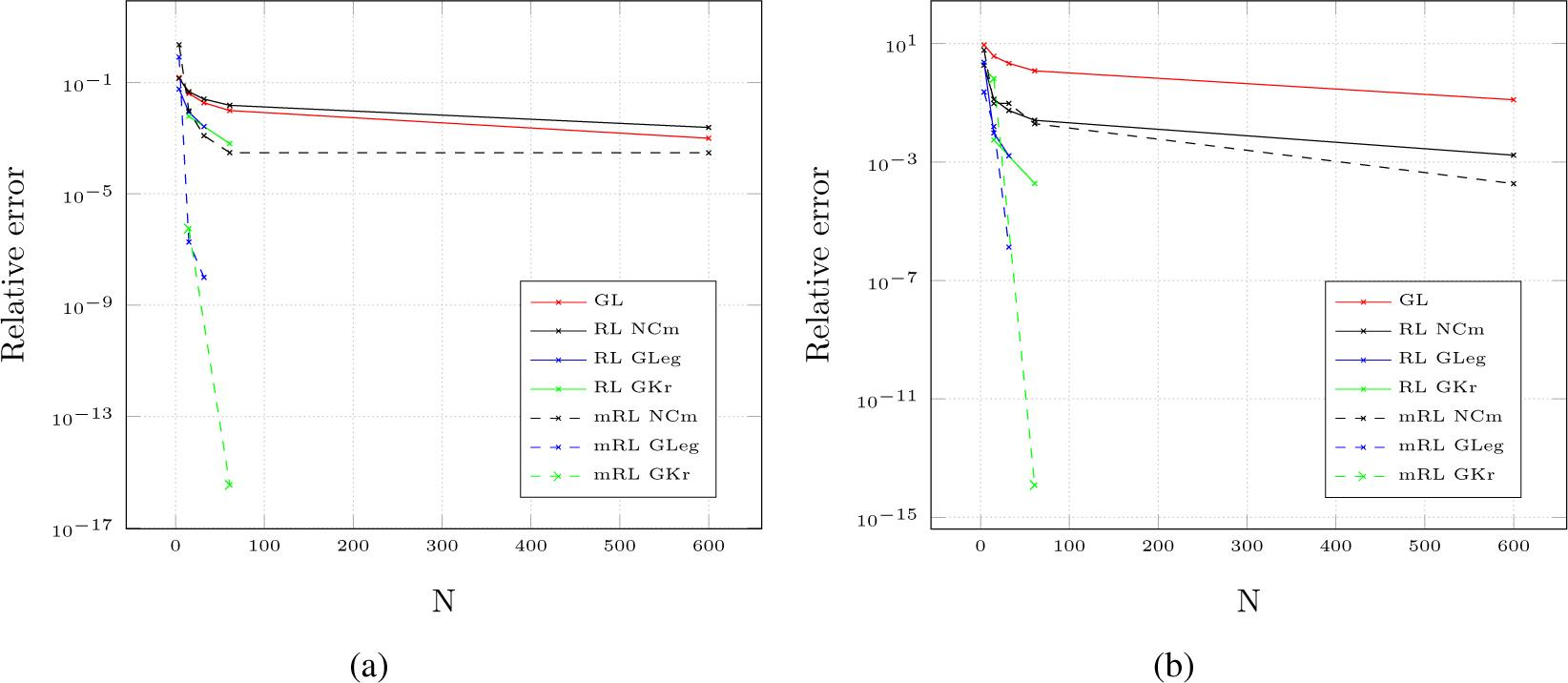Computational accuracy of fractional derivative of order ν = 0.5 for the function (11)(a) and (12)(b) applying unmodified(RL ...) and modified(mRL ...) formula (5).

Final remark:

Analytical independent variable substitution in the integrand is a very effective method of accuracy increase. An average four times accuracy increase of the transformed integrand integration proves it. Application of the technique improves efficiency as well; the increased accuracy is obtained by using half of the sampling points required by the same numerical integration method applied to the unchanged integrand.

How high the accuracy increase actually is depends on the shape of the transformed integrand and how much it agrees with an “optimal” shape for high accuracy integration by applying particular method of integration.

Nevertheless, “manual” transformation is arduous and time consuming, which can hinder some applications.

Integrand Transformation as a Numerical Quadrature. The Double Exponential Transformation

Transformation of an integrand via independent variable substitution can also be applied in form of a numerical quadrature. Such approach enables to omit arduous analytical transformation of each integrand and automate the whole transformation process.

An efficient and universal method of an integrand transformation is known as the Double Exponential Transformation (DE). The DE Transformation is a joint of hyperbolic function independent variable substitution in the integrand and the Trapezoidal Rule.

Such transformed form will be referred as the Double Exponential Quadrature (the DE Quadrature).

The general idea of this kind of variable transformation was proposed for the first time by Korbov . It also became a subject of works of Schwartz , Stenger , Takahasi , Mori , Haber  and others, who gave it different names.

The DE transformation never achieved a wider popularity due to poor publicity and some drawbacks. The drawbacks are mainly associated with the limitations of the single and double precision arithmetic applied in the initial programming at the time of development, e.g. limited range of applied single and double precision variables which can cause under- and over-flow when calculating weights required for the quadrature.

Two solutions of this problem, which involve another substitution at the singularity point have been published in , ,  and lately in .

Unfortunately, application of the solutions decrease overall accuracy abilities of the method.

The problem can also be solved by applying arbitrary precision variables and arbitrary precision mathematical library GNU MPFR, which improves accuracy of the DE Transformation.

In the following section, the DE Transformation is applied to calculate fractional orders derivatives and integrands applying formulas (5) and (6).

The DE Transformation implemented for the purpose of fractional orders derivatives and integrands computations is based on the transformation, which was proposed by Schwartz  as the Tanh Rule. It can be described as follows:

Let us consider the integral

$S=∫abf(x)dx,$$$\begin{array}{} \displaystyle S = \int_a^b f \left( x \right)dx, \end{array}$$

where f(x) is integrable on interval (a,b). The function f(x) may have singularity at x = a or x = b or at both ends.

First we apply the following variable transformation

$x=ϕ(t), ϕ(−∞)=a, ϕ(∞)=b.$$$\begin{array}{} \displaystyle x = \phi \left( t \right),\;\phi \left( { - \infty } \right) = a,\;\phi \left( \infty \right) = b. \end{array}$$

We obtain

$S=∫−∞∞f(ϕ(t))ϕ′(t)dt.$$$\begin{array}{} \displaystyle S = \int _{ - \infty }^\infty f\left( {\phi \left( t \right)} \right)\phi \prime \left( t \right)dt. \end{array}$$

It is important that ϕ(t) possesses the property such as ϕ′(t) converges at least at single exponential rate as t → ±∞

$|ϕ′(t)|→exp(−cexp(|t|)),$$$\begin{array}{} \displaystyle \left| {\phi \prime \left( t \right)} \right| \to \exp \left( { - c\exp \left( {\left| t \right|} \right)} \right), \end{array}$$

where c is some constant. After that, it is best to apply the Trapezoidal Rule to the transformed integrand expression , 

$S=h∑n=−∞∞f(ϕ(nh))ϕ′(nh),$$$\begin{array}{} \displaystyle S = h\mathop \sum \limits_{n = - \infty }^\infty f\left( {\phi \left( {nh} \right)} \right)\phi' \left( {nh} \right), \end{array}$$

where h is subinterval width. Due to the property (16) truncation of the summation process can be done at some arbitrarily chosen n = −N and n = +N

$S=h∑n=−N+Nf(ϕ(nh))ϕ′(nh),$$$\begin{array}{} \displaystyle S = h\mathop \sum \limits_{ + N}^{n = - N} f\left( {\phi \left( {nh} \right)} \right)\phi \prime \left( {nh} \right), \end{array}$$

$N=−N+N+1,$$$\begin{array}{} \displaystyle N = - N + N + 1, \end{array}$$

where N states the amount of subintervals.

Since ϕ′(nh) as well as the whole expression f(ϕ(nh))ϕ′ (nh) converges at least at exponential rate at large |n|, the quadrature formula (17) is called the Double Exponential .

Due to truncation of the summation process in (17) at some arbitrary chosen n = −N, n = +N, function f(x) can have singularities at x = a and/or x = b as long as it is integrable over the integration interval.

Two kinds of errors should be taken into consideration when implementing the DE Formula: discretization error, due to the use of the Trapezoidal Rule to approximate an integral and truncation error, because of truncation of infinite sum at some N. The optimal strategy is to get both errors equal , .

The subinterval width h, that defines the evaluation step and the number of sampling points are key values in such strategy. The source  suggests the following value of h for the DE Formula

$h~log(2πNω/c)N,$$$\begin{array}{} \displaystyle h \sim \frac{{\log (2\pi N\omega /c)}}{N}, \end{array}$$

where c is some constant to be taken, usually 1 or π/2 and ω is the distance to the nearest singularity of the integrand defined in , .

The following equations (18)-(20) present three different transformations with varied convergence rate , . Transformation (18) has the slowest convergence and transformation (20), the fastest respectively:

$x=ϕ(t)=tanh tp, ϕ′(t)=ptp−1cosh2tp, p=1,3,5,…,$$$\begin{array}{} \displaystyle x = \phi \left( t \right) = \tanh \,{t^p},\,\phi \prime \left( t \right) = \frac{{p{t^{p - 1}}}}{{{{\cosh }^2}{t^p}}},\,p = 1,3,5, \ldots , \end{array}$$

$x=ϕ(t)=tanh(π2sinh t), ϕ′(t)=π2 cosh tcosh2(π2 sinh t),$$$\begin{array}{} \displaystyle x = \phi \left( t \right) = \tanh \left( {\frac{\pi }{2}\sinh \,t} \right),\,\phi \prime \left( t \right) = \frac{{\frac{\pi }{2}\,\cosh \,t}}{{{{\cosh }^2}\left( {\frac{\pi }{2}\,\sinh \,t} \right)}}, \end{array}$$

$x=ϕ(t)=tanh(π2sinh t3), ϕ′(t)=32π t2 cosh t3cosh2(π2 sinh t3).$$$\begin{array}{} \displaystyle x = \phi \left( t \right) = \tanh \left( {\frac{\pi }{2}\sinh \,{t^3}} \right),\,\phi \prime \left( t \right) = \frac{{\frac{3}{2}\pi \,{t^2}\,\cosh \,{t^3}}}{{{{\cosh }^2}\left( {\frac{\pi }{2}\,\sinh \,{t^3}} \right)}}. \end{array}$$

Applying the transformation (19) to a function according to the formula (17), we obtain the following trapezoidal form

$S=h∑i=1Nf(b−a2 xi+b+a2)wi,$$$\begin{array}{} \displaystyle S = h\mathop \sum \limits^N_{i = 1} f\left( {\frac{{b - a}}{2}\,{x_i} + \frac{{b + a}}{2}} \right){w_i}, \end{array}$$

where

$xi=f(tanh(π2 sinh ti)),$$$\begin{array}{} \displaystyle {x_i} = f\left( {\tanh \left( {\frac{\pi }{2}\;\sinh \;{t_i}} \right)} \right), \end{array}$$

$ti=−ta+(i−1)h, i=1,2,3,⋯N,h=2taN,$$$\begin{array}{} \displaystyle {t_i} = - {t_a} + \left( {i - 1} \right)h,\;i = 1,2,3, \cdots N,h = \frac{{2{t_a}}}{N}, \end{array}$$

$wi=π2 cosh ticosh2(π2sinh ti)⋅(b−a2)$$$\begin{array}{} \displaystyle {w_i} = \frac{{\frac{\pi }{2}\;\cosh \;{t_i}}}{{{{\cosh }^2}\left( {\frac{\pi }{2}\sinh \;{t_i}} \right)}} \cdot \left( {\frac{{b - a}}{2}} \right) \end{array}$$

are the nodes, the sampling points and the weights of the DE Quadrature respectively.

Parameter ta can be calculated using the following formula 

$$\begin{array}{} \displaystyle {t_a} = {\rm{precision}}{\rm{s digit}}{{\rm{s}}^{0.46}}, \end{array}$$

According to  asymptotic error of the formula (21) in terms of subinterval width h of the Trapezoidal Rule is expressed as

$|ΔSh|≈exp(−ch),$$$\begin{array}{} \displaystyle \left| {\Delta {S_h}} \right| \approx \exp \left( { - \frac{c}{h}} \right), \end{array}$$

and asymptotic error in terms of the number N of the functions evaluations is

$|ΔSh(N)|≈exp(−cNlogN).$$$\begin{array}{} \displaystyle \left| {\Delta S_h^{\left( N \right)}} \right| \approx \exp \left( { - c\frac{N}{{\log N}}} \right). \end{array}$$

Programming Issues

Calculation of the DE Quadrature weights from the formula (21) can cross the double precision variables limits. First, the denominator in the weight function formula sec2 (csinh t) can overflow. Second, the selection of the parameter ta > 3 (22) can cause underflow and the occurrence of uncontrolled errors (the computed integral values replaced by random numbers).

Increasing value of the parameter ta we can integrate further left and right from y axis (see Fig. 5(c)) and it increases the accuracy. However, values of integrated function can assume very small values. Too small for double precision variables to distinguish them from 0. The underflow occurs. This negative process can be eliminated by applying high precision variables. Application of GNU MPFR library makes it possible. The library have also at their disposal many mathematical functions which can be used with the high precision variables.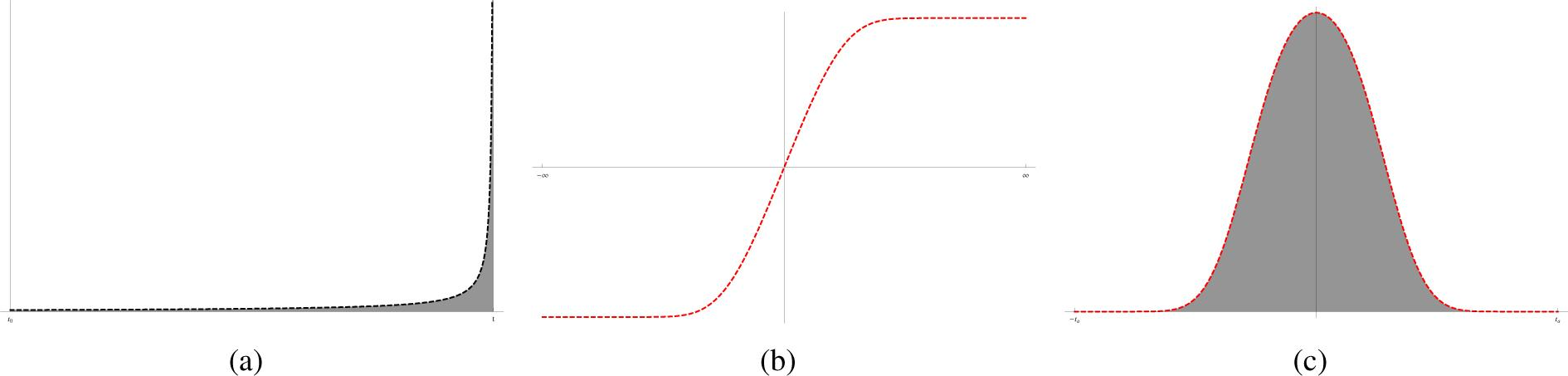The Double Exponential Transformation: (a) graph of the original integrand,(b) graph of transforming expression and (c) transformed integrand.

For ta = 4.97 there are required 100-digits variables, for ta = 5.5 - 200-digits variables, for ta = 6.5 - 500-digits variables, for ta = 7.5 - 1500-digits variables and for ta = 8.7 - 5000-digits variables.

Another parameter which influence accuracy of the DE Quadrature (21) is an amount N of subintervals for integration applying the Trapezoidal Rule.

Accuracy of the DE Transformation for Fractional Derivatives and Integrals Computations

For accuracy assessment of the DE Transformation there are selected three functions:

$f(t)=e−t, t∈(0,5),$$$\begin{array}{} \displaystyle f\left( t \right) = {e^{ - t}},\;t \in \left( {0,5} \right), \end{array}$$

$f(t)=sin(t), t∈(0,2π),$$$\begin{array}{} \displaystyle f\left( t \right) = \sin \left( t \right),\;t \in \left( {0,2\pi } \right), \end{array}$$

$f(t)=t 1(t), t∈(0,1)$$$\begin{array}{} \displaystyle f\left( t \right) = t\;{\bf{1}}\left( t \right),\;t \in \left( {0,1} \right) \end{array}$$

and four fractional orders:

Fractional derivative of order: ν = 0.9 and ν = 0.5

Fractional integral of order: ν = −0.1 and ν = −0.0001,

Results are presented in Figs. 6, 7 and 8.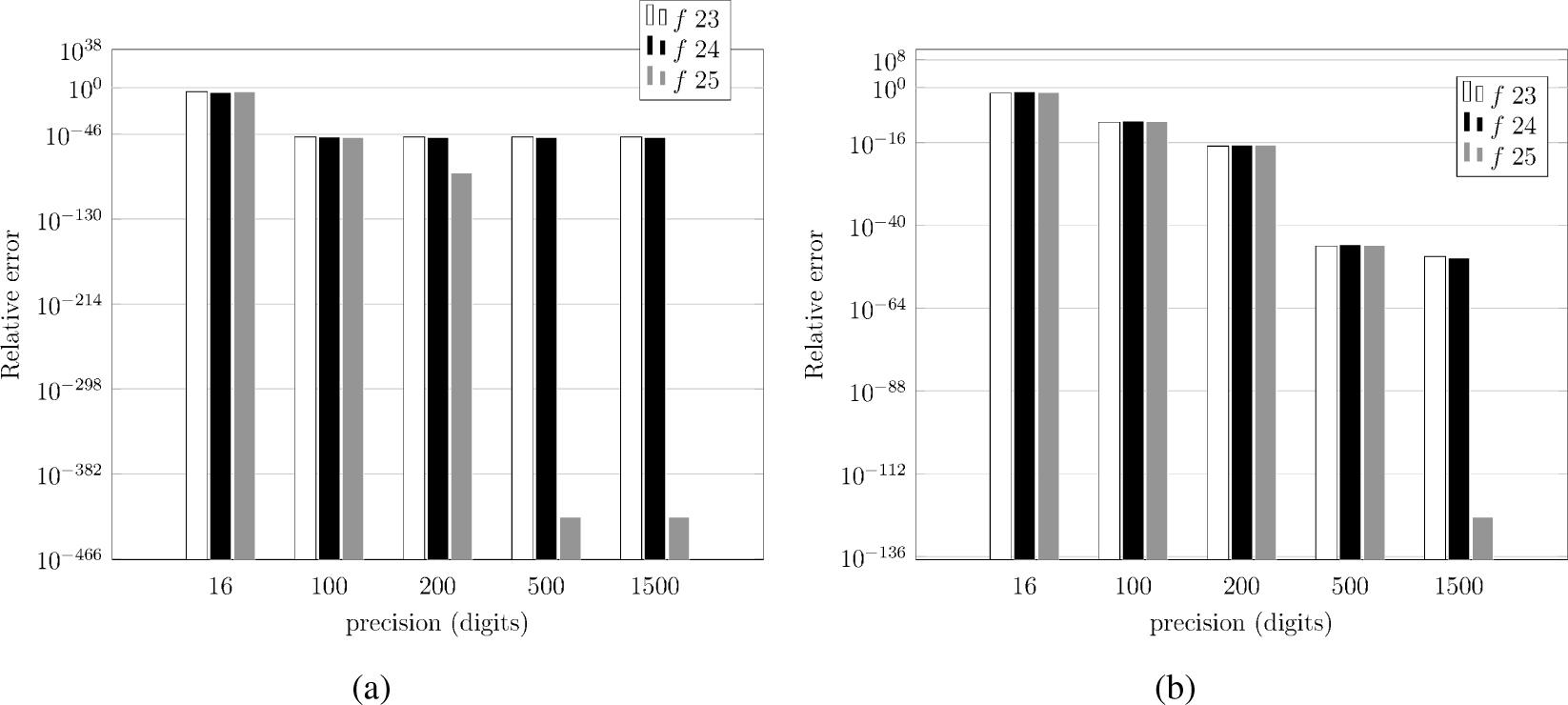Accuracy of the DE Transformation for orders ν = 0.9(a), ν = 0.5(b) and N=1000 in context of applied precision during computations.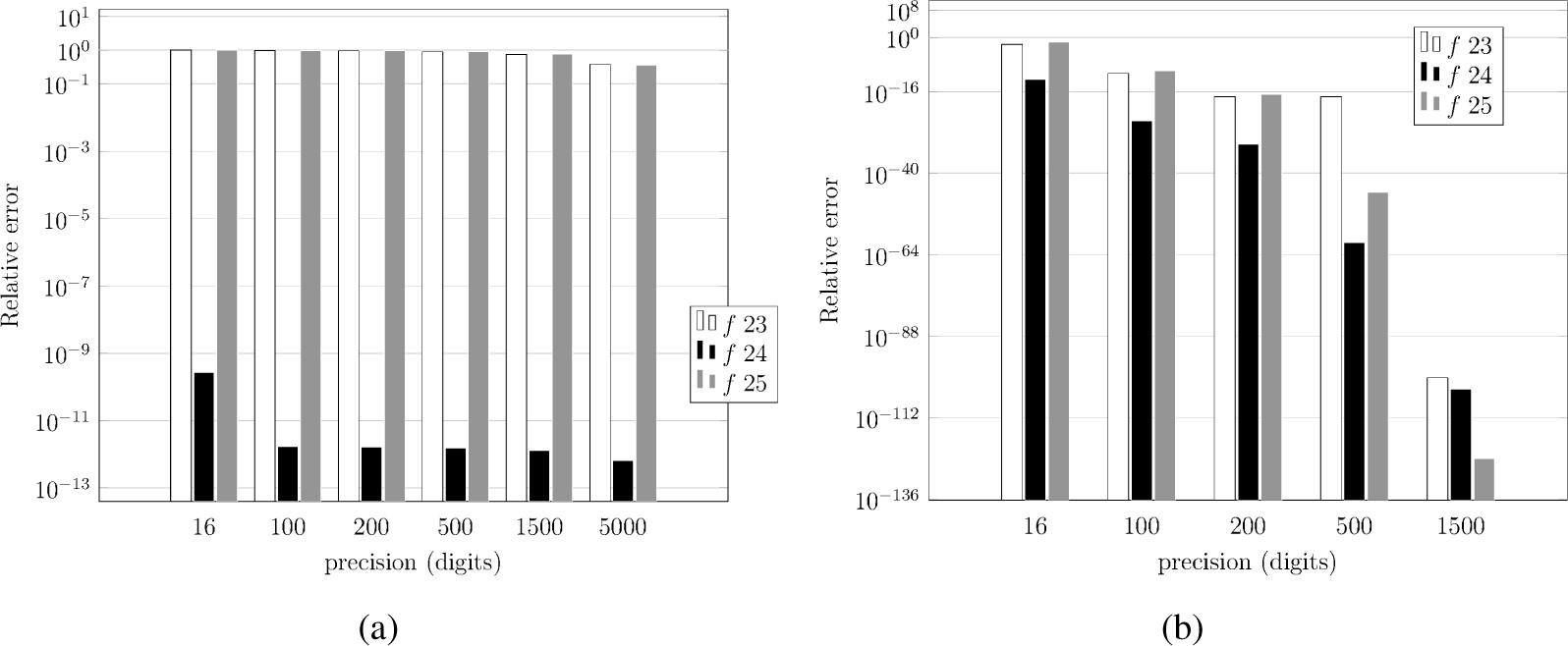Accuracy of the DE Transformation for orders ν = −0.0001(a), ν = −0.1(b) and N=1000 in context of applied precision during computations.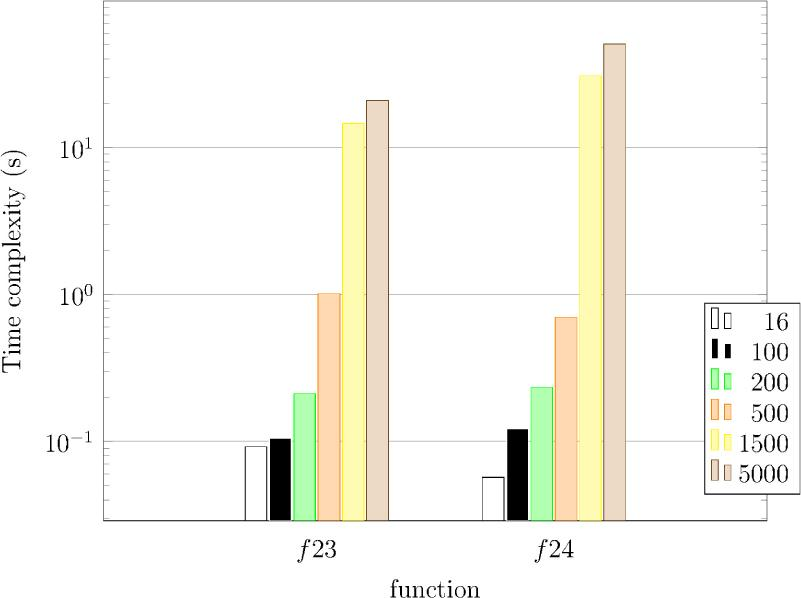Time complexity for increasing precision (digits), N=1000, fractional integral ν = −0.0001 for the function (26) and ν = 0.9 for the function (27).

Final remarks:

Independent variable substitution in integrand in a form of a numerical quadrature has even more advantages than its “manual” counterpart presented in the previous subsection: it can be applied automatically during the integration, the nodes and the weights of the DE Quadrature can be computed only once and applied many times. Their computation is not difficult.

How high the accuracy increase actually can be depends on the shape of the transformed integrand and how much it agrees with an “optimal” shape for high accuracy integration by applying the Trapezoidal Rule and how “far” left and right from the y axis it is possible to integrate. The latter is difficult due to very small values occurrence on both ends of the transformed integration interval which cause underflows applying standard precision in computations.

The accuracy increase up to ∼ 10−50 can be obtained by careful programming applying variables with increased precision. Still, some orders are out of reach as for example the order ν = −0.0001, calculation of which ends up with 90% relative error.

The reason for the unsatisfactory accuracy for the orders near 0 and 1 is the decreasing value of power in the denominator in the Riemann-Liouville/Caputo formulas and limitations of the applied substitution expression.

Using the DE Transformation with the double precision, the accuracy is limited to two decimal places.

Despite the obvious advantages of arbitrary precision application, the user must be aware that they extend immensely time required for computations if amount of precision digits applied in calculations is greater that 100 (see Fig. 8).

The Gauss-Jacobi Quadrature

There exists a wide range of numerical methods for solutions of singularities and infinite limits issues in numerical integration, e.g. the Gauss Quadratures. They involve application of polynomials in an integral approximation. The polynomials’ properties are selected for the specific integration problem and thereby they assure high accuracy results. The problematic area for integration is computed differently, which assures high accuracy.

Generally, the Gauss Quadratures can be applied to a specific types of integrands only. It is conditioned by the linkage to their weight functions (with exclusion of the Gauss-Legendre Quadrature with the weight function equals 1).

The Gauss Quadratures are commonly applied to compute most difficult integrals, e.g. the improper integrals and integrals with end point singularities.

The ordinarily sufficient method of the Gauss Quadratures application is reduced to the use of tabulated values of nodes and weights. They are freely available ,  and easy to incorporate into a computer program. However, the integration process can become difficult if nodes and weights must be computed, an integrand does not meet exactly or at all requirements of an quadrature’s weight function or an integration interval is different than assumed.

The first case requires the application of mathematical formulas for nodes and weights.

The second issue solution involves manual extraction of a weight function from an integrand or a weight function adjustment to a problematic integrand.

Changing integration interval from default to arbitrary selected involves a conversion if a quadrature’s requirements permit it.

Next there are presented some important details to the Gauss-Jacobi Quadrature numerical application requirements as well as the formulas for the nodes and the weights computations.

A weight function which eliminates definite integration range endpoints singularities is Jacobi weight function

$p(x)≡(1−x)λ(1+x)β, λ,β>−1.$$$\begin{array}{} \displaystyle p\left( x \right) \equiv {\left( {1 - x} \right)^\lambda }{(1 + x)^\beta },\;\lambda ,\beta \gt - 1. \end{array}$$

General, well known formula of approximate calculation of definite integral

$∫abp(x)f(x)dx≅∑k=1nAkf(xk)+R$$$\begin{array}{} \displaystyle \int_a^b p \left( x \right)f\left( x \right)dx \cong \sum\limits_{k = 1}^n {{A_k}} f\left( {{x_k}} \right) + R \end{array}$$

with the weight function (26) assumes the form:

$∫−11(1−x)λ(1+x)β⋅f(x)dx≈∑k=1nAkf(xk),$$$\begin{array}{} \displaystyle \int_{ - 1}^1 {{{\left( {1 - x} \right)}^\lambda }} {(1 + x)^\beta } \cdot f\left( x \right)dx \approx \sum\limits_{k = 1}^n {{A_k}} f\left( {{x_k}} \right), \end{array}$$

in which xk are zeros of Jacobi polynomial $Jn(λ,β)(xk)$$\begin{array}{} \displaystyle J_n^{\left( {\lambda ,\beta } \right)}\left( {{x_k}} \right) \end{array}$ of degree n.

The Jacobi Polynomial can be determined by applying Rodrigues formula

$Jn(λ,β)(x)=(−1)n2n⋅n!(1−x)−λ(1+x)−βdndxn[(1−x)λ+n(1+x)β+n]$$$\begin{array}{} \displaystyle J_n^{\left( {\lambda ,\beta } \right)}\left( x \right) = \frac{{{{\left( { - 1} \right)}^n}}}{{2n \cdot n!}}{\left( {1 - x} \right)^{ - \lambda }}{\left( {1 + x} \right)^{ - \beta }}\frac{{{d^n}}}{{d{x^n}}}\left[ {{{\left( {1 - x} \right)}^{\lambda + n}}{{\left( {1 + x} \right)}^{\beta + n}}} \right] \end{array}$$

and weights

$Ak=2λ+β+1Γ(λ+n+1)Γ(β+n+1)n!Γ(λ+β+n+1)1(1−xk2)[Jn(λ,β)′xk]2,$$$\begin{array}{} \displaystyle {A_k} = {2^{\lambda + \beta + 1}}\frac{{\Gamma \left( {\lambda + n + 1} \right)\Gamma \left( {\beta + n + 1} \right)}}{{n!\Gamma \left( {\lambda + \beta + n + 1} \right)}}\frac{1}{{\left( {1 - x_k^2} \right){{\left[ {J_n^{\left( {\lambda ,\beta } \right)\prime }{x_k}} \right]}^2}}}, \end{array}$$

in which $Jn′(xk)$$\begin{array}{} \displaystyle J_n^\prime \left( {{x_k}} \right) \end{array}$ is derivative $Jn(λ,β)′(xk)$$\begin{array}{} \displaystyle J_n^{\left( {\lambda ,\beta } \right)\prime }\left( {{x_k}} \right) \end{array}$ of Jacobi polynomial of degree n and

$R=2λ+β+2n+1λ+β+2n+1Γ(λ+n+1)Γ(β+n+1)Γ(λ+β+n+1)Γ2(λ+β+2n+1)n!2nf2n(ξ), ξ∈〈−1,1〉=O(n2)$$$\begin{array}{} \displaystyle R = \frac{{{2^{\lambda + \beta + 2n + 1}}}}{{\lambda + \beta + 2n + 1}}\frac{{\Gamma \left( {\lambda + n + 1} \right)\Gamma \left( {\beta + n + 1} \right)\Gamma \left( {\lambda + \beta + n + 1} \right)}}{{{\Gamma ^2}\left( {\lambda + \beta + 2n + 1} \right)}}\frac{{n!}}{{2n}}{f^{2n}}\left( \xi \right),\,\xi \in \langle - 1,1\rangle = {\scr O}({n^2}) \end{array}$$

is the remainder of integral approximation.

Approximation of Fractional Derivatives and Integrals

Substituting with λ = 1 − ν,β = 0 in the weight formula (29) we obtain

$∫−11f(t)(1−t)1−νdt≈∑k=1nAkf(tk)=Σk=1n2ν(1−tk2)[Jn(ν−1,0)′tk]2f(tk)$$$\begin{array}{} \displaystyle \int _{ - 1}^1\frac{{f\left( t \right)}}{{{{\left( {1 - t} \right)}^{1 - \nu }}}}dt \approx \mathop \sum \limits^n_{k = 1} {A_k}f\left( {{t_k}} \right) = \mathop {\rm{\Sigma }}\limits^n_{k = 1} \frac{{{2^\nu}}}{{\left( {1 - t_k^2} \right){{[J_n^{\left( {\nu - 1,0} \right)\prime }{t_k}]}^2}}}f\left( {{t_k}} \right) \end{array}$$

and relation 

$∑k=1n2ν(1−tk2)[Jn(ν−1,0)′(tk)]2∫−11(1−t)1−νJn(ν−1,0)(t)(t−tk)·Jn(ν−1,0)′(tk)dt,$$$\begin{array}{} \displaystyle \mathop \sum\limits^n_{k = 1} \frac{{{2^\nu}}}{{\left( {1 - t_k^2} \right){{\left[ {J_n^{\left( {\nu - 1,0} \right)\prime }\left( {{t_k}} \right)} \right]}^2}}}\int _{ - 1}^1{\left( {1 - t} \right)^{1 - \nu}}\frac{{J_n^{\left( {\nu - 1,0} \right)}\left( t \right)}}{{\left( {t - {t_k}} \right)\ . J_n^{\left( {\nu - 1,0} \right)\prime }\left( {{t_k}} \right)}}dt, \end{array}$$

Transforming the interval [t0,t] into [−1,1], the integral $∫t0tf(t)(t−τ)1−νdτ$$\begin{array}{} \displaystyle \int_{{t_0}}^t {\frac{{f\left( t \right)}}{{{{\left( {t - \tau } \right)}^{1 - \nu }}}}} d\tau \end{array}$ can be rewritten as

$(t−t02)ν∫−11f(u)(1−u)1−νdu$$$\begin{array}{} \displaystyle {\left( {\frac{{t - {t_0}}}{2}} \right)^\nu }\int_{ - 1}^1 {\frac{{f\left( u \right)}}{{{{\left( {1 - u} \right)}^{1 - \nu }}}}} du \end{array}$$

in which

$f(u)=f((t−t02)u+(t+t02)).$$$\begin{array}{} \displaystyle f\left( u \right) = f\left( {\left( {\frac{{t - {t_0}}}{2}} \right)u + \left( {\frac{{t + {t_0}}}{2}} \right)} \right). \end{array}$$

Applying (31) we can express Riemann-Liouville fractional order integral formula (6) as

$t0RLDt(−ν)f(t)=1Γ(ν)(t−t02)ν∫−11f(u)(1−u)1−νdu=1Γ(ν)(t−t02)νΣk=1n2ν(1−uk2)[Jn(ν−1,0)′uk]2 f(uk).$$$\begin{array}{} \displaystyle _{{t_0}}^{RL}{\rm{D}}_t^{\left( { - \nu } \right)}f\left( t \right) = \frac{1}{{\Gamma \left( \nu \right)}}{\left( {\frac{{t - {t_0}}}{2}} \right)^\nu }\int _{ - 1}^1\frac{{f\left( u \right)}}{{{{\left( {1 - u} \right)}^{1 - \nu}}}}du = \frac{1}{{\Gamma \left( \nu \right)}}{\left( {\frac{{t - {t_0}}}{2}} \right)^\nu}\mathop {\rm{\Sigma }}\limits^n_{k = 1} \frac{{{2^\nu}}}{{\left( {1 - u_k^2} \right){{\left[ {J_n^{\left( {\nu - 1,0} \right)\prime }{u_k}} \right]}^2}}}\,f\left( {{u_k}} \right). \end{array}$$

To calculate fractional order derivatives we proceed the similar way

$∑k=0n−1(t−t0)k−νf(k)(t0)Γ(k−ν+1)+1Γ(n−ν)(t−t02)n−ν∫−11f(n)(u)(1−u)ν−n+1du$$$\begin{array}{} \displaystyle \sum\limits_{k = 0}^{n - 1} {\frac{{{{\left( {t - {t_0}} \right)}^{k - \nu }}{f^{\left( k \right)}}\left( {{t_0}} \right)}}{{\Gamma \left( {k - \nu + 1} \right)}}} + \frac{1}{{\Gamma \left( {n - \nu } \right)}}{\left( {\frac{{t - {t_0}}}{2}} \right)^{n - \nu }}\int_{ - 1}^1 {\frac{{{f^{\left( n \right)}}\left( u \right)}}{{{{\left( {1 - u} \right)}^{\nu - n + 1}}}}} du \end{array}$$

where

$f(n)(u)=f(n)((t−t02)u+(t+t02)).$$$\begin{array}{} \displaystyle {f^{\left( n \right)}}\left( u \right) = {f^{\left( n \right)}}\left( {\left( {\frac{{t - {t_0}}}{2}} \right)u + \left( {\frac{{t + {t_0}}}{2}} \right)} \right). \end{array}$$

The Riemann-Liouville fractional order derivative formula (5) assumes the following form

$t0RLDtνft=∑k=0n−1t−t0k−νfkt0Γk−ν+1+1Γn−ν⋅t−t02n−ν∫−11fnu1−uν−n+1du=∑k=0n−1t−t0k−νfkt0Γk−ν+1+1Γn−ν⋅t−t02n−ν∑k=1n2ν1−uk2Jnν−n+1,0′uk2fnuk.$$$\begin{multline} ^{RL}_{t_{0}}\textrm{D}_{t}^{\left ( \nu \right )}f\left(t\right)=\sum_{k=0}^{n-1}\frac{ \left(t-t_{0}\right)^{k-\nu} f^{\left( k \right)}\left ( t_{0} \right )}{\Gamma\left ( k-\nu+1 \right )} +\frac{1}{\Gamma\left ( n-\nu \right )} \cdot \left ( \frac{t-t_{0}}{2} \right )^{n-\nu}\int_{-1}^{1}\frac{ f^{\left(n\right)} \left(u\right)}{\left ( 1-u \right )^{\nu-n+1}}du \\ = \sum_{k=0}^{n-1}\frac{ \left(t-t_{0}\right)^{k-\nu} f^{\left( k \right)}\left ( t_{0} \right )}{\Gamma\left ( k-\nu+1 \right )} +\frac{1}{\Gamma\left ( n-\nu \right )} \cdot \left ( \frac{t-t_{0}}{2} \right )^{n-\nu} \sum_{k=1}^{n} \frac{2^{\nu}}{ \left( 1-u^{2}_{k} \right) \left[ J_{n}^{\left(\nu-n+1,0\right){'}} u_{k} \right]^{2}}\;f^{\left(n\right)}\;\left(u_{k}\right). \end{multline}$$

in which n = ⌈ν⌉.

Programming Issues

The Gauss-Jacobi Quadrature (27) requires computation of polynomial of order n, its derivative, nodes and weights.

Existing approaches to nodes and weights computing, some of which have been widely used for many years, suffer from 𝒪(n2) complexity or error which grows with n. With some optimizations, e.g. application of technique which utilizes asymptotic formulas for accurate initial guesses of the roots and efficient evaluations of the degree n Jacobi polynomial (28), it can be reduced to 𝒪(n) .

Accuracy of the Gauss-Jacobi Quadrature for Fractional Order Derivatives and Integrals Computations

Accuracy of the method is conducted in the similar conditions as in the case of DE Transformation. First, there is assessed accuracy for double precision (denoted as 16-digits precision) and high precision calculations. Wherein, it is only required to 100-digit variables increase in high precision calculations for GJ to achieve the highest possible accuracy for the method. Hence the lack of results for other precision options present in the DE Transformation accuracy assessment. Results are presented in Figs. 9 and 10.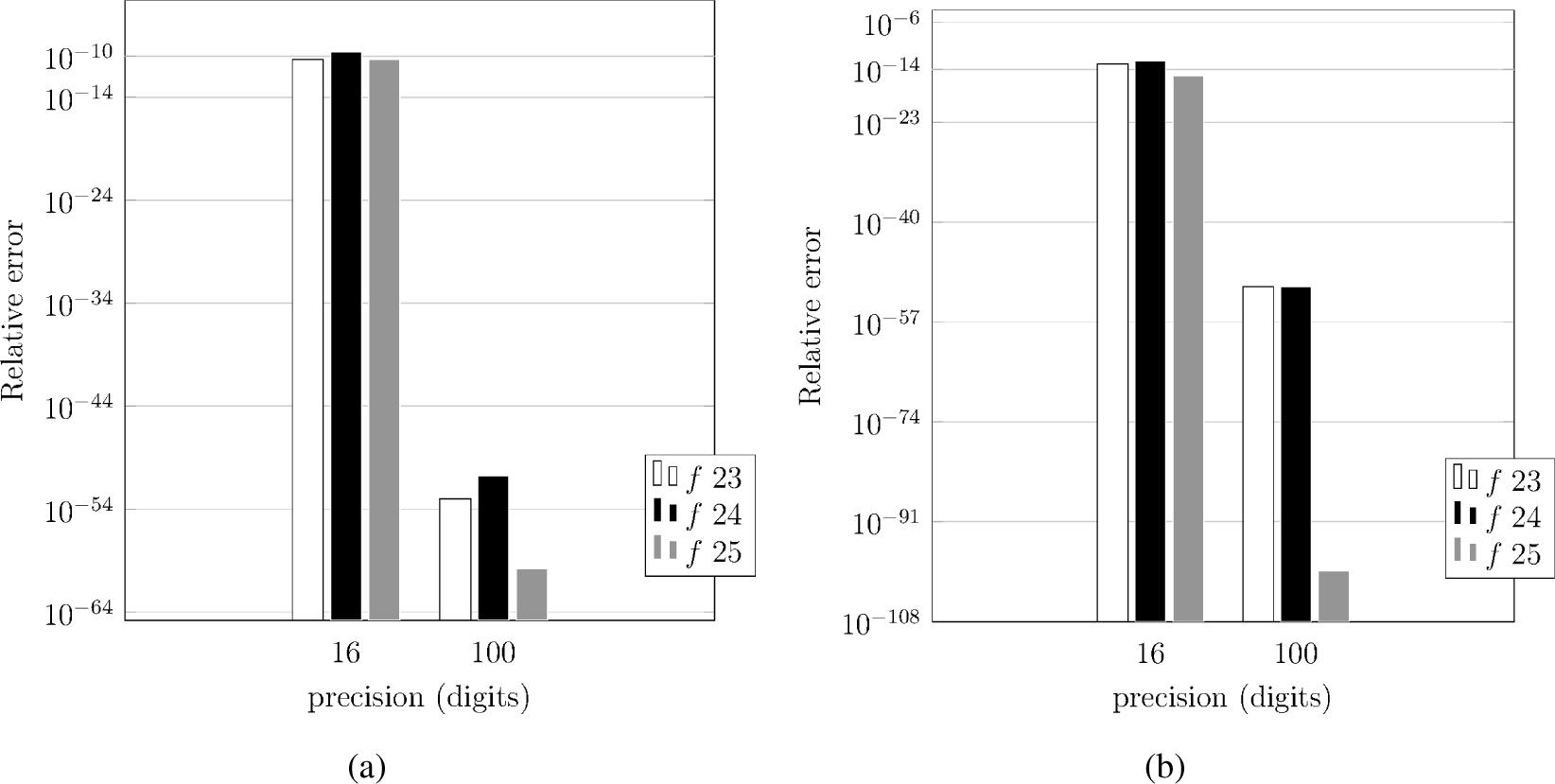Accuracy of GJ with N = 32 for calculating fractional integrals of orders ν = −0.0001(a) and ν = −0.1(b); 16 vs 100 digits precision.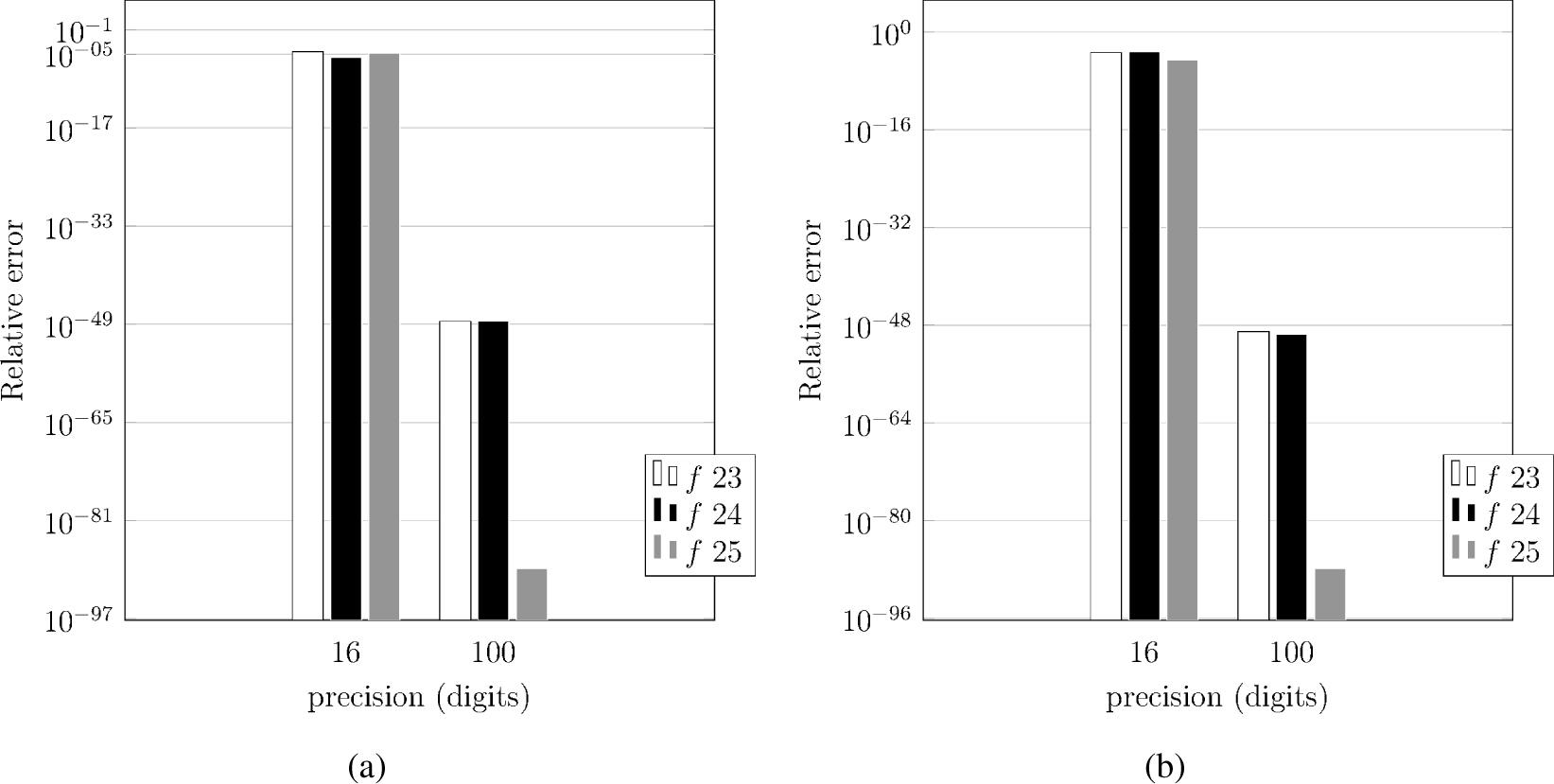Accuracy of GJ with N = 32 for calculating fractional derivatives of orders ν = 0.5(a) and ν = 0.9(b); 16 vs 100 digits precision.

The Gauss Jacobi Quadrature is applied for calculations of fractional order integrals ν = −0.0001, ν = −0.1 and derivatives ν = 0.5, ν = 0.9 of exponential function (23), trigonometric function (24) and the function (25).

Next, GJ is evaluated with N = 32 degree of polynomial applied in integral approximation. The value is an outcome from the results of the next part accuracy assessment of the method, i.e. comparison of accuracy performance of GJ for various degree of polynomial. It is presented in Figs. 11 and 12.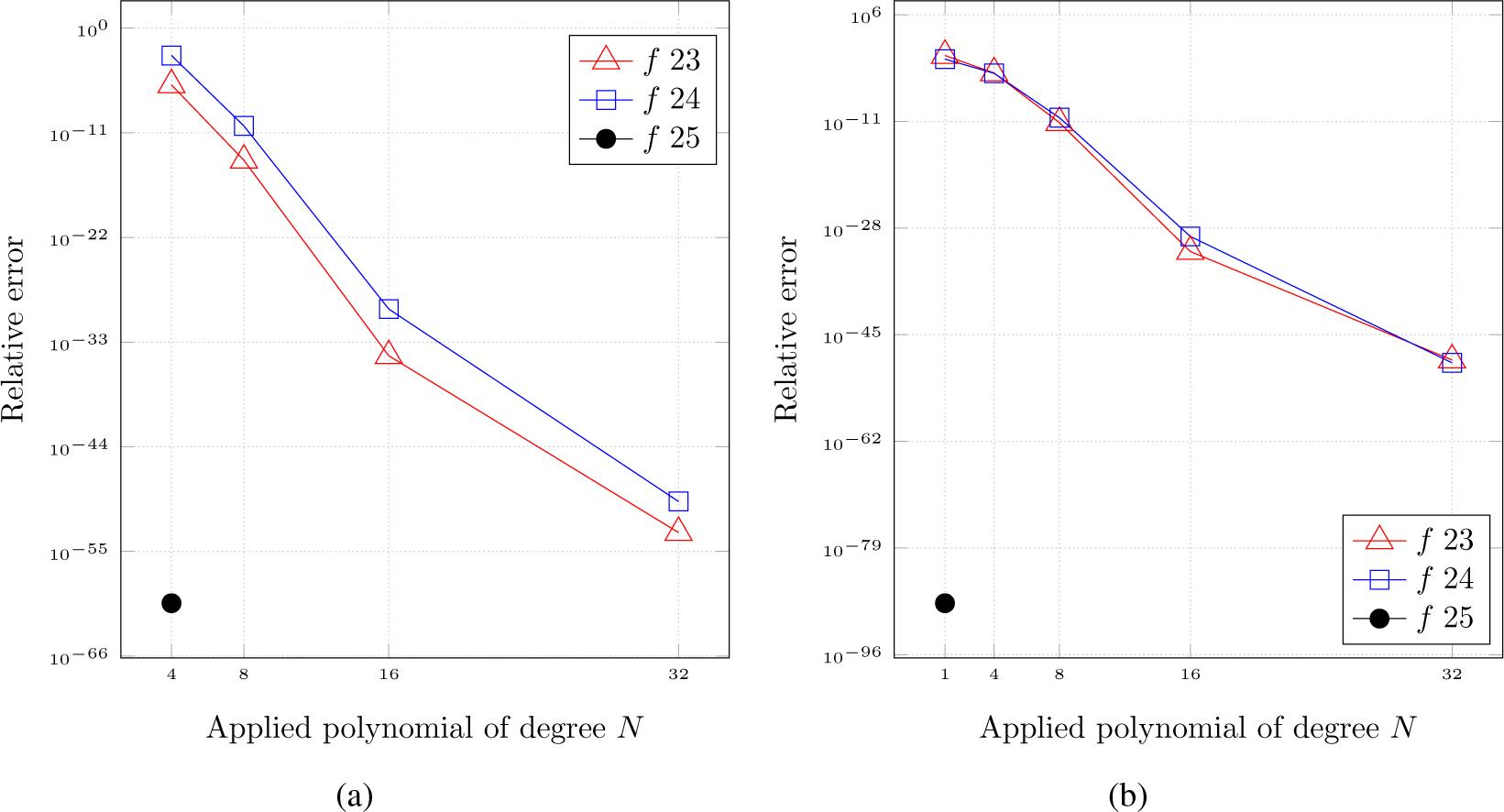Accuracy of GJ for calculating fractional integrals of orders ν = −0.0001(a) and ν = 0.9(b). N = 4,8,16,32 with 100 digits precision.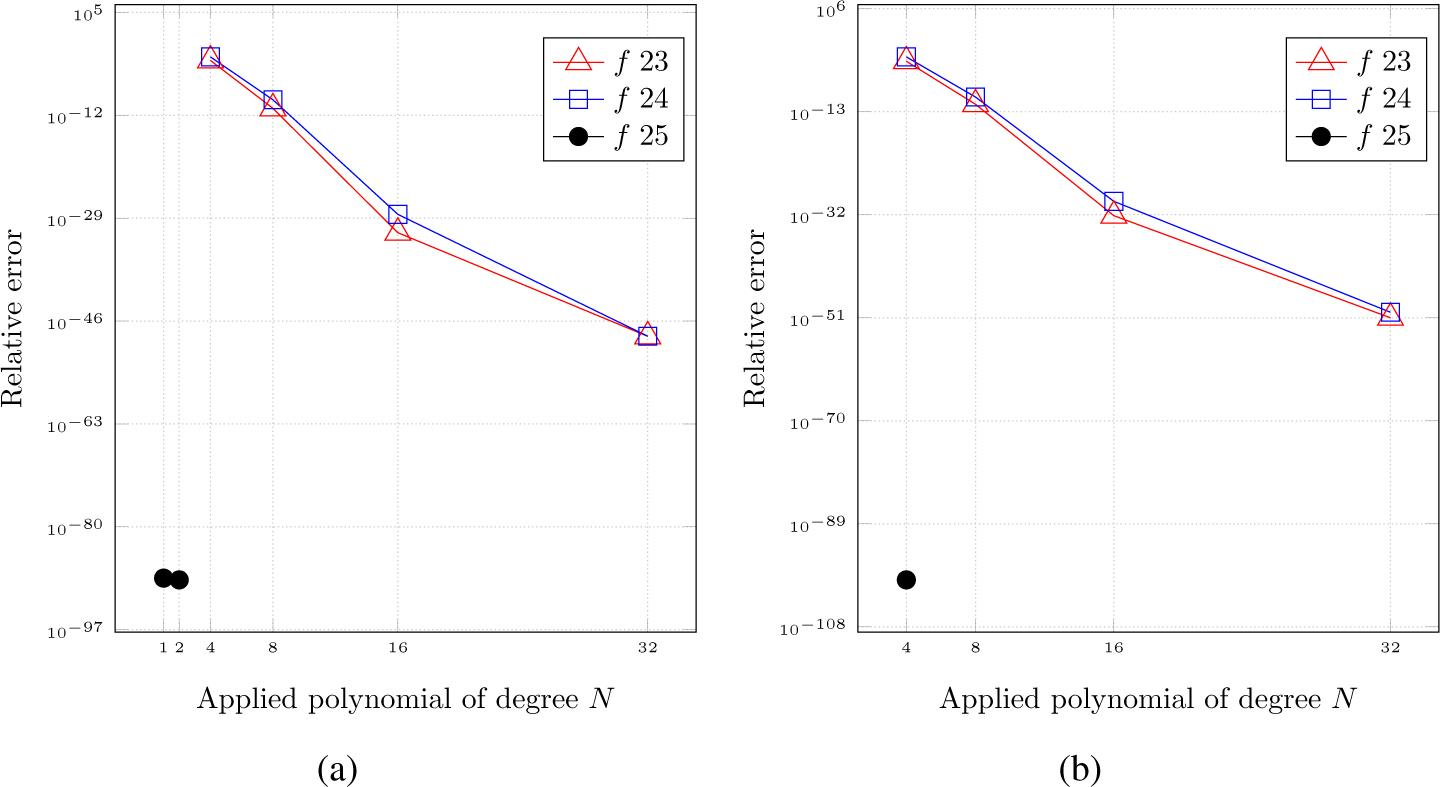Accuracy of GJ for calculating fractional derivatives of orders ν = 0.5(a) and ν = 0.9(b). N = 1,2,4,8,16,32 with 100 digits precision.

Now, GJ is evaluated for accuracy of calculations for different fractional orders 0 < ν < 1 and different integration intervals (1(1)6). Results are presented in Figs. 13.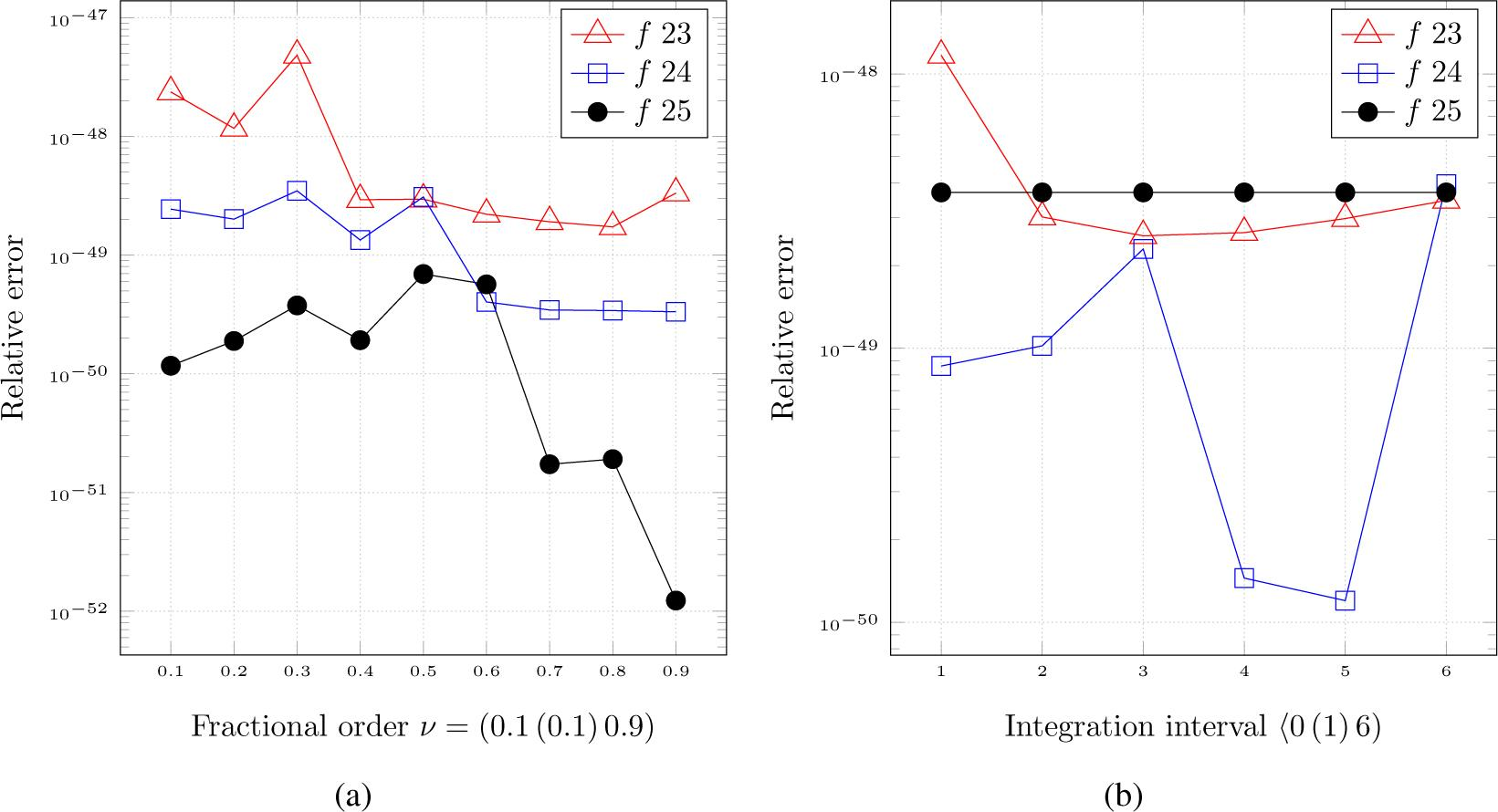Accuracy of GJ for calculating fractional derivatives of orders (0.1(0.1)0.9) (a) and of order ν = 0.9999 for intervals (1(1)6) (b), N = 32 with 100 digits precision.

Fig. 14 presents comparison of rough time complexity of calculations for GJ, DE Transformation (DE) and Grünwald-Letnikov method (GL) applied with double and 100-digits precision.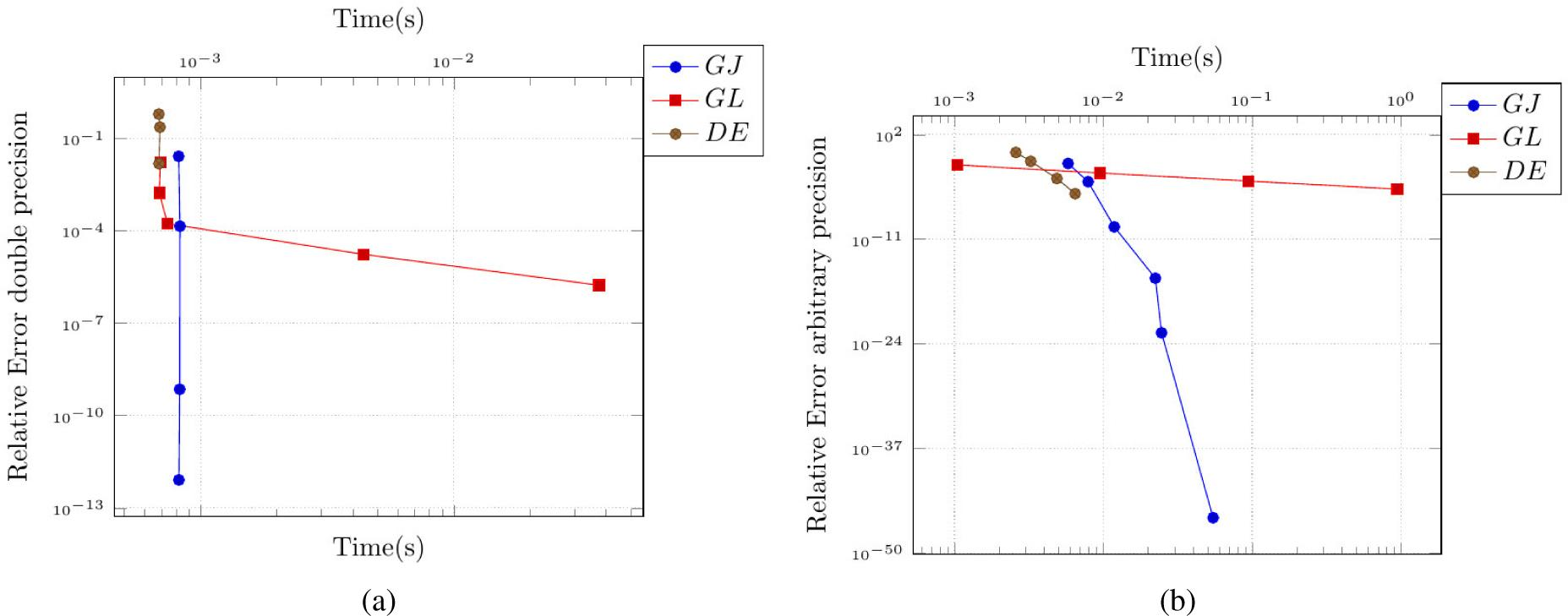Programs running time for the function (23), (a) programmed with double precision and (b) with 100-digits precision.

Final remarks:

Approximation of fractional order derivatives and integrals applying the Gauss-Jacobi Quadrature is the most effective method of accuracy increase from all presented in the paper. The integrand is left intact and instead, the weights are changed according to its properties; the computation of the difficult kernel in the Riemann-Liuoville/Caputo formulas is removed from the integration (it is now calculated using the weight function). Programming the quadrature applying variables with increased precision ascertain constant high accuracy of computations, independently from integrated function, fractional order and interval. Wherein difficult fractional orders of integrals near 0 and fractional orders of derivatives near 1, which are out of reach for any other methods can also be calculated with similar high accuracy.

The degree N of polynomial applied in integral approximation and an amount of digits of precision used for calculation are the only factors which influence calculation accuracy.

Another advantage of the method is that application of high order polynomial N = 32 and 100-digit precision does not extend significantly time complexity of calculations (see Fig. 14).

Conclusions

The problems associated with singularities are the most difficult to solve in numerical integration. However, application of a proper transformation to the integrand in the Riemann-Liouville/Caputo formulas or a correct selection of parameters for the weight function of the Gauss-Jacobi Quadrature make possible to calculate fractional order derivatives and integrals with high-accuracy. The accuracy gain can be improved by application of arbitrary precision in computations.

Analytical integrand transformation applying appropriate in context of a selected method of integration substitution expression is very effective and can increase accuracy of numerical integration significantly. However, the method is arduous because it requires manual transformation of each integrand.

Transformation of the integrand via independent variable substitution applied in a form of a numerical quadrature enables to omit arduous analytical transformation of an integrand and automate the whole substitution process.

Application of the DE Quadrature turned the integration process into automatic one. It is effective under the condition of finding and applying substitution expression which transforms an integrand into one which matches optimal requirements of the Trapezoidal Rule.

Approximation of fractional order derivatives and integrals by applying the Gauss-Jacobi Quadrature is the most effective method which assures high-accuracy results up to ∼ 10−120 mark independently from fractional order, integration interval and a type of a function.Plot of the kernel of the integrand in the formulas (3), (5) and (6).(a) Graph of the original integrand g(τ), (b) graph of transformed variable τ into u and (c) transformed integrand g(τ) into G(u).Computational accuracy of fractional integral of order ν = −0.5 for the function (11)(a) and (12)(b) applying unmodified(RL ...) and modified(mRL ...) formula (6).Computational accuracy of fractional derivative of order ν = 0.5 for the function (11)(a) and (12)(b) applying unmodified(RL ...) and modified(mRL ...) formula (5).The Double Exponential Transformation: (a) graph of the original integrand,(b) graph of transforming expression and (c) transformed integrand.Accuracy of the DE Transformation for orders ν = 0.9(a), ν = 0.5(b) and N=1000 in context of applied precision during computations.Accuracy of the DE Transformation for orders ν = −0.0001(a), ν = −0.1(b) and N=1000 in context of applied precision during computations.Time complexity for increasing precision (digits), N=1000, fractional integral ν = −0.0001 for the function (26) and ν = 0.9 for the function (27).Accuracy of GJ with N = 32 for calculating fractional integrals of orders ν = −0.0001(a) and ν = −0.1(b); 16 vs 100 digits precision.Accuracy of GJ with N = 32 for calculating fractional derivatives of orders ν = 0.5(a) and ν = 0.9(b); 16 vs 100 digits precision.Accuracy of GJ for calculating fractional integrals of orders ν = −0.0001(a) and ν = 0.9(b). N = 4,8,16,32 with 100 digits precision.Accuracy of GJ for calculating fractional derivatives of orders ν = 0.5(a) and ν = 0.9(b). N = 1,2,4,8,16,32 with 100 digits precision.Accuracy of GJ for calculating fractional derivatives of orders (0.1(0.1)0.9) (a) and of order ν = 0.9999 for intervals (1(1)6) (b), N = 32 with 100 digits precision.Programs running time for the function (23), (a) programmed with double precision and (b) with 100-digits precision.

#### D(1/2)f(t), f(t) = t, t ∈ (0,1), relative error in %

NGLNCmDietOdiba
88.1110.680.00040.0024
154.257.810.00040.0023
213.026.60.00040.0023
611.033.880.00040.0022
3000.211.750.00020.0021
6000.111.240.00020.0021
10000.060.960.00020.0021

NGLNCm
861.8713.52
1529.325.88
2120.174.12
616.541.81
3001.30.76
6000.650.53
10000.390.41

#### D(1/2)f(t), f(t) = sin(t), t ∈ (0,2π), relative error in %

NGLNCmDietOdiba
8130.0345.4217.675.78
1566.1433.647.953.46
2146.0928.775.182.94
6116.117.841.532.94
3005.029.350.622.43
6003.677.290.562.35
10003.146.180.552.35

R. Herrmann. Fractional Calculus. An Introduction for Physicists, 2nd. ed. World Scientific, Singapore, 2014. 10.1080/00107514.2011.648957HerrmannR.Fractional Calculus. An Introduction for Physicists2ndWorld ScientificSingapore201410.1080/00107514.2011.648957

G. W. Leibniz. Letter from hannover, germany, to g. f. a. l’hôpital, september 30. 1695. In Mathematische Schriften, 1849, pages 301–302. Olms Verlag, Hildesheim, Germany, 1695. Reprint 1962.LeibnizG. W.Letter from hannover, germany, to g. f. a. l’hôpitalseptember 30. 1695In Mathematische Schriften1849301302Olms Verlag, HildesheimGermany16951962Search in Google Scholar

L. Debnath and D. Bhatta. Integral Transforms and Their Applications, Third Edition. CRC Press, Taylor & Francis Group, Boca Raton London New York, 2015. 10.1007/978-1-4684-9283-5DebnathL.BhattaD.Integral Transforms and Their ApplicationsThird EditionCRC Press, Taylor & Francis GroupBoca Raton London New York201510.1007/978-1-4684-9283-5

B. Ross. Proceedings to the international conference on fractional calculus and its applications. June 1975. 10.1007/BFb0067095RossB.Proceedings to the international conference on fractional calculus and its applicationsJune197510.1007/BFb0067095

K. Oldham and J. Spanier. The Fractional Calculus. Theory and Applications of Differentiation and Integration to Arbitrary Order. Academic Press, INC, San Diego Ca, 1974.OldhamK.SpanierJ.The Fractional CalculusTheory and Applications of Differentiation and Integration to Arbitrary OrderAcademic Press, INCSan Diego Ca1974Search in Google Scholar

Guillaume Hanrot et al. mpfr: The MPFR library for multiple-precision floating-point computations with correct rounding. (version 3.13), 2015. http://www.mpfr.org/.HanrotGuillaumeet almpfr: The MPFR library for multiple-precision floating-point computations with correct rounding. (version 3.13)2015http://www.mpfr.org/Search in Google Scholar

Torbjörn Granlund et al. gmp: GMP is a free library for arbitrary precision arithmetic (version 6.0.0a), 2015. https://gmplib.org/.GranlundTorbjörnet algmp: GMP is a free library for arbitrary precision arithmetic (version 6.0.0a)2015https://gmplib.org/Search in Google Scholar

K. S. Miller and B. Ross. An Introduction to the Fractional Calculus and Fractional Differential Equations. John Wiley & Sons, Inc., New York, NY, 1993.MillerK. S.RossB.An Introduction to the Fractional Calculus and Fractional Differential EquationsJohn Wiley & Sons, IncNew York, NY1993Search in Google Scholar

M. D. Ortigueira, J. A. T. Machado, and J. Sa da Costa. Which differintegration? IEE Proceedings - Vision, Image and Signal Processing, 152(6), 2005.OrtigueiraM. D.MachadoJ. A. T.Sa da CostaJ.Which differintegration?IEE Proceedings - Vision, Image and Signal Processing15262005Search in Google Scholar

S. Samko, A. Kilbas, and O. Marichev. Fractional Integrals and Derivatives: Theory and Applications. Gordon and Breach, London, 1993.SamkoS.KilbasA.MarichevO.Fractional Integrals and Derivatives: Theory and ApplicationsGordon and BreachLondon1993Search in Google Scholar

J. T. Machado. Numerical calculation of the left and right fractional derivatives. Journal of Computational Physics, 293:96–103, 2015. 10.1016/j.jcp.2014.05.029MachadoJ. T.Numerical calculation of the left and right fractional derivativesJournal of Computational Physics29396103201510.1016/j.jcp.2014.05.029

I. Podlubny. Fractional Differential Equations. Academic Press, INC, San Diego Ca, 1999.PodlubnyI.Fractional Differential EquationsAcademic Press, INCSan Diego Ca1999Search in Google Scholar

Piotr Ostalczyk. Zarys rachunku ró˙zniczkowego i całkowego ułamkowych rze¸dów. Wydawnictwo Politechniki Łódzkiej, Łódź, Poland, 2006.OstalczykPiotrZarys rachunku ró˙zniczkowego i całkowego ułamkowych rze¸dówWydawnictwo Politechniki Łódzkiej, ŁódźPoland2006Search in Google Scholar

D. Valerio, J. J. Trujillo, M. Rivero, J. A. T. Machado, and D. Baleanu. Fractional calculus: A survey of useful formulas. The European Physical Journal Special Topics, 222:1827–1846, 2013. 10.1140/epjst/e2013-01967-yValerioD.TrujilloJ. J.RiveroM.MachadoJ. A. T.BaleanuD.Fractional calculus: A survey of useful formulasThe European Physical Journal Special Topics22218271846201310.1140/epjst/e2013-01967-y

M. D. Ortigueira. Fractional central differences and derivatives. Journal of Vibration and Control, 14(9-10):1255–1266, 2008. 10.1177/1077546307087453OrtigueiraM. D.Fractional central differences and derivativesJournal of Vibration and Control149-1012551266200810.1177/1077546307087453

Piotr Ostalczyk. Discrete Fractional Calculus. Applications in Control and Image Processing. World Scientific, 2016. 10.1142/9789814725675OstalczykPiotrDiscrete Fractional Calculus. Applications in Control and Image ProcessingWorld Scientific201610.1142/9789814725675

P. K. Kythe and M. R. Schaferkotter. Handbook of Computational Methods for Integration. Chapman & Hall/CRC, 2005.KytheP. K.SchaferkotterM. R.Handbook of Computational Methods for IntegrationChapman & Hall/CRC2005Search in Google Scholar

R. L. Burden and J.D. Faires. Numerical Analysis, 5th ed. Brooks/Cole Cengage Learning, Boston, 2003. 10.2307/2348648BurdenR. L.FairesJ.D.Numerical Analysis5thBrooks/Cole Cengage LearningBoston200310.2307/2348648

B.P. Demidowicz, I.A. Maron, and E.Z. Szuwałowa. Metody Numeryczne. Państwowe Wydawnictwo Naukowe, Warszawa, Poland, 1965.DemidowiczB.P.MaronI.A.SzuwałowaE.Z.Metody NumerycznePaństwowe Wydawnictwo NaukoweWarszawa, Poland1965Search in Google Scholar

S. C. Chapra and R. P. Canale. Numerical Methods for Engineers, 6th Ed. McGraw-Hill Companies, Inc., 2010. 10.1036/0072431938ChapraS. C.CanaleR. P.Numerical Methods for Engineers6thMcGraw-Hill Companies, Inc201010.1036/0072431938

M. Hjorton-Jensen. Computational Physics. University of Oslo, Oslo, Norway, 2009.Hjorton-JensenM.Computational PhysicsUniversity of OsloOslo, Norway2009Search in Google Scholar

J. Kiusalaas. Numerical Methods in Engineering With Python. Cambridge University Press, 2005.KiusalaasJ.Numerical Methods in Engineering With PythonCambridge University Press2005Search in Google Scholar

K. Diethelm and G. Walz. Numerical solution of fractional order differential equations by extrapolation. Numerical Algorithms, 16:231–253, 1997. 10.1023/A:1019147432240DiethelmK.WalzG.Numerical solution of fractional order differential equations by extrapolationNumerical Algorithms16231253199710.1023/A:1019147432240

K. Diethelm, N.J. Ford, A.D. Freed, and Yu. Luchko. Algorithms for the fractional calculus: A selection of numerical methods. Comput. Methods Appl. Engrg., 194:743–773, 2005. 10.1016/j.cma.2004.06.006DiethelmK.FordN.J.FreedA.D.LuchkoYu.Algorithms for the fractional calculus: A selection of numerical methodsComput. Methods Appl. Engrg194743773200510.1016/j.cma.2004.06.006

Z. Odibat. Approximation of fractional integrals and caputo fractional derivatives. Applied Mathematics and Computation, 178:527–533, 2006. 10.1016/j.amc.2005.11.072OdibatZ.Approximation of fractional integrals and caputo fractional derivativesApplied Mathematics and Computation178527533200610.1016/j.amc.2005.11.072

D. A. Murio. On the stable numerical evaluation of caputo fractional derivatives. An International Journal: Computers & Mathematics with Applications, 51:1539–1550, 2006. 10.1016/j.camwa.2005.11.037MurioD. A.On the stable numerical evaluation of caputo fractional derivativesAn International Journal: Computers & Mathematics with Applications5115391550200610.1016/j.camwa.2005.11.037

H. Sugiura and T. Hasegawa. Quadrature rule for abel’s equations: Uniformly approximating fractional derivatives. Journal of Computational and Applied Mathematics, 223(1):459–468, 2009. 10.1016/j.cam.2008.01.019SugiuraH.HasegawaT.Quadrature rule for abel’s equations: Uniformly approximating fractional derivativesJournal of Computational and Applied Mathematics2231459468200910.1016/j.cam.2008.01.019

H. Bateman. Tables of Integral Transforms, vol. II. Mc Graw-Hill Book Company, Inc., New York, Tornoto, London, 1954. 10.1021/ed031p392.4BatemanH.Tables of Integral Transforms, vol. IIMc Graw-Hill Book Company, IncNew York, Tornoto, London195410.1021/ed031p392.4

D. W. Brzeziński and P. Ostalczyk. Accuracy assessment of fractional order derivatives and integrals numerical computations. Journal of Applied Nonlinear Dynamics, 4(1):53–65, 2015. 10.5890/JAND.2015.03.005BrzezińskiD. W.OstalczykP.Accuracy assessment of fractional order derivatives and integrals numerical computationsJournal of Applied Nonlinear Dynamics415365201510.5890/JAND.2015.03.005

N. M. Korobov. Teoretikocislwyje metody w priblizennom analize. Gosudarstwennoe Izdatelstwo FizykoMatematiceskoj Literatury, Mockba, 1963.KorobovN. M.Teoretikocislwyje metody w priblizennom analizeGosudarstwennoe Izdatelstwo FizykoMatematiceskoj LiteraturyMockba1963Search in Google Scholar

C. Schwartz. Numerical integration of analytic functions. Journal of Computational Physics, 4:19–29, 1969. 10.1063/1.4756325SchwartzC.Numerical integration of analytic functionsJournal of Computational Physics41929196910.1063/1.4756325

F. Stenger. Integration formulae based on the trapezoidal formula. J. Inst. Math. Appl., 12:103–114, 1973. 10.1093/imamat/12.1.103StengerF.Integration formulae based on the trapezoidal formulaJ. Inst. Math. Appl12103114197310.1093/imamat/12.1.103

H. Takahashi. Quadrature formulas obtained by variable transformation. Numer. Math, 21:206–219, 1973. 10.1007/BF01436624TakahashiH.Quadrature formulas obtained by variable transformationNumer. Math21206219197310.1007/BF01436624

M. Mori. Developments in the double exponential formulas for numerical integration. In Proceedings of the International Congress of Mathematicians, pages 1585–1594, Kyoto, Japan, 1990.MoriM.Developments in the double exponential formulas for numerical integrationIn Proceedings of the International Congress of Mathematicians15851594Kyoto, Japan1990Search in Google Scholar

S. Haber. The tanh rule for numerical integration. J. Numer. Anal., 14:668–685, 1999. 10.1137/0714045HaberS.The tanh rule for numerical integrationJ. Numer. Anal14668685199910.1137/0714045

H. Takahashi and M. Mori. Double exponential formulas for numerical integration. Publ. RIMS, 9:721–744, 1974. 10.2977/prims/1195192451TakahashiH.MoriM.Double exponential formulas for numerical integrationPubl. RIMS9721744197410.2977/prims/1195192451

T. Watanabe. On the double exponential formula for numerical integration. Kakuyugokenkyu, 63(5):397–411, 1990.WatanabeT.On the double exponential formula for numerical integrationKakuyugokenkyu6353974111990Search in Google Scholar

W. H. Press, S. A. Teukolsky, W. T. Vetterling, and B. P. Flannery. Numerical Recipes. The Art of Scientific Computing, Third Edition. Cambridge University Press, 2007. 10.1007/BF01321860PressW. H.TeukolskyS. A.VetterlingW. T.FlanneryB. P.Numerical Recipes. The Art of Scientific ComputingThird EditionCambridge University Press200710.1007/BF01321860

J. Waldvogel. Towards a general error theory of the trapezoidal rule. Approximation and Computation, 42:267–282, 2011. 10.1007/978-1-4419-6594-3_17WaldvogelJ.Towards a general error theory of the trapezoidal ruleApproximation and Computation42267282201110.1007/978-1-4419-6594-3_17

M. Abramowitz and I. A. Stegun. Handbook of Mathematical Functions. Applied Mathematics Series. Cambridge University Press, 1968. 10.2307/2314682AbramowitzM.StegunI. A.Handbook of Mathematical FunctionsApplied Mathematics SeriesCambridge University Press1968doi 10.2307/2314682

V. I. Krylov. Priblizhennoe wychislenie integralov, 2e izd. Nauka, Mockba, 1967.KrylovV. I.Priblizhennoe wychislenie integralov, 2e izdNauka, Mockba1967Search in Google Scholar

N. Hale and A. Townsend. Fast and accurate computation of gauss-legendre and gauss-jacobi quadrature nodes and weights. Oxford Centre for Collaborative Applied Mathematics, 2012. OCCAM Preprint Number 12/79. 10.1007/s12346-015-0136-1HaleN.TownsendA.Fast and accurate computation of gauss-legendre and gauss-jacobi quadrature nodes and weightsOxford Centre for Collaborative Applied Mathematics, 2012. OCCAM Preprint Number 12/7910.1007/s12346-015-0136-1